Journal topic
Solid Earth, 11, 767–789, 2020
https://doi.org/10.5194/se-11-767-2020Solid Earth, 11, 767–789, 2020
https://doi.org/10.5194/se-11-767-2020Research article 05 May 2020

Research article | 05 May 2020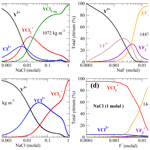# Yttrium speciation in subduction-zone fluids from ab initio molecular dynamics simulations

Yttrium speciation in subduction-zone fluids from ab initio molecular dynamics simulations
Johannes Stefanski and Sandro Jahn Johannes Stefanski and Sandro Jahn
• Institute of Geology and Mineralogy, University of Cologne, Zülpicher Str. 49b, 50674 Cologne, Germany

Correspondence: Sandro Jahn (s.jahn@uni-koeln.de)

Abstract

The rare Earth elements (REEs) are important geochemical tracers for geological processes such as high-grade metamorphism. Aqueous fluids are considered important carriers for the REEs in a variety of geological environments including settings associated with subduction zones. The capacity of a fluid to mobilize REEs strongly depends on its chemical composition and on the presence of suitable ligands such as fluoride and chloride. In this study, we present structural and thermodynamic properties of aqueous yttrium–chloride and yttrium–fluoride species at a temperature of 800 C in a pressure range between 1.3 and 4.5 GPa derived from ab initio molecular dynamics simulations. The total yttrium coordination by H2O and halide ions changes from seven to eight within the pressure range. For the yttrium–chloride species, a maximum number of three chloride ligands was observed. The derived thermodynamic data show that aqueous yttrium–fluoride complexes are more stable than their yttrium–chloride counterparts in chloride- and fluoride-rich environments at conditions relevant to slab dehydration. Mixed Y(Cl,F) complexes are found to be unstable even on the molecular dynamics timescale. Furthermore, in contrast to field observations, thermodynamic modeling indicates that yttrium should be mobilized at rather low fluoride concentrations in high-grade metasomatic systems. These results suggest a rather low fluoride activity in the majority of subduction-zone fluids because yttrium is one of the least-mobile REEs. Additionally, the simulations indicate that yttrium drives the self-ionization of hydration water molecules as it was observed for other high-field-strength elements. This might be a general property for highly charged cations in aqueous solutions under high-temperature and high-pressure conditions.

1 Introduction

Subduction zones have been the most important sites for exchange of matter and energy between the Earth's crust and mantle for billions of years until now . Magnetotelluric anomalies (e.g., ) suggest the occurrence of a high proportion of melts and water-rich fluids in the subducted slabs due to partial melting and the dehydration of water-bearing minerals such as serpentine and amphibole . Quite naturally, these fluids do not consist of pure water; much rather, they are brines with high salinity up to several mass percent of Cl and contain Si, Al and alkali cations (Na, K) as major solutes with minor amounts of Ca, Fe and Mg .

Aqueous fluids play an important role in subduction zones. Their interaction with minerals and rocks results in alterations including the dissolution and precipitation of minerals and/or the exchange of chemical elements and isotopes. Furthermore, fluid-mediated transport of trace elements such as high-field-strength elements (HFSEs) and rare Earth elements (REEs) is a major process of the deep element cycles (Manning2004). The distribution of these elements between minerals and fluids or melts is used as petrogenetic indicator for fractionation processes in igneous and metasomatic petrology (Winter2009). It is known that REE patterns of subducted rocks are affected by the chemical composition of the metamorphic fluid (e.g., ) due to their chemical complexation with dissolved anions, e.g., F, ${\mathrm{SO}}_{\mathrm{4}}^{\mathrm{2}-}$, ${\mathrm{CO}}_{\mathrm{3}}^{\mathrm{2}-}$ and Cl .

The speciation of REEs at moderate pressures (up to few 100 MPa) and high temperatures (250–300 C) was studied experimentally using in situ X-ray absorption spectroscopy (XAS) and solubility experiments (see a recent review by ) to understand physicochemical properties of hydrothermal fluids related to REE ore deposition focusing on chloride and fluoride complexes. Due to the high stability of fluoride complexes , it is a widely shared notion that fluoride complexes are most important for REE transport in hydrothermal fluids, but suggested that REE fluoride complexes are not the major carrier of REEs due to the low solubility of REE fluoride minerals such as bastnaesite, $\left(\mathrm{Ce},\mathrm{La},\mathrm{Nd},\mathrm{Y}\right)\left[{\mathrm{FCO}}_{\mathrm{3}}\right]$, and due to the low fluoride activity in low pH environments. According to , REE chloride and sulfate complexes appear to be the main species for REE transport in hydrothermal systems. However, this interpretation is questioned by other authors .

So far, the number of in situ studies that address the complexation or thermodynamic properties of REE aqueous species at pressure (P) and temperature (T) conditions of subduction zones is very limited due to the challenging experimental setups . Apart from field observations (e.g., fluid inclusion analysis), our main understanding of the behavior of REEs under high PT conditions is derived from fluid/mineral partitioning and solubility experiments (e.g., ) and from numerical simulations. In a case study, modeled the hydration shell of REEs in solution by static energy calculations of an explicit first hydration shell and an implicit solvent model. Temperature effects were introduced by considering changes of the dielectric constant of the solvent and by calculations of the entropy. In this study, it is concluded that the hydration energies of all lanthanides become more similar at high PT conditions and that the availability of ligands becomes a controlling factor for the fractionation of light REEs (LREEs) and heavy REEs (HREEs) by subduction-zone fluids. Experiments suggest that LREEs (e.g., La) are more mobile than HREEs in chloride-rich solutions . The presence of fluoride in the system enhances the mobility of HREEs and this leads to fractionation processes .

From a geochemical perspective, yttrium is considered a HREE1, and as such it is very common to use yttrium as a representative of the whole group of HREEs because of their similar chemical properties. Further, the comparable behavior of Y and the majority of the HREEs in high-grade metasomatism processes (Ague2017) supports this assumption. The hydration shell of Y3+ in aqueous solutions and possible complexation of Y3+ with chloride has been subject to a number of studies at room temperature (e.g., ). Molecular simulations in conjunction with advanced sampling methods indicate that in the absence of other ligands Y3+ is coordinated by eight hydration water molecules , whereas at high pH [Y(OH)3(H2O)3]aq complexes are formed . The reported Y–O distance of 2.38 Å agrees with extended X-ray absorption fine structure (EXAFS) and X-ray absorption near edge structure (XANES) measurements .

The complexation of Y3+ with Cl was studied by at hydrothermal conditions up to 340 C using in situ EXAFS spectroscopy. The authors claim that yttrium is coordinated by eight to nine neighbors at hydrothermal conditions and does not associate with chloride but rather forms polyatomic yttrium species. In another study by , a strong association of Y with chloride up to ${\mathrm{YCl}}_{\mathrm{4}}^{-}$ at 500 C and a linear reduction of the total number of coordinating atoms from eight to four towards high temperatures are reported. The results of that study indicate that yttrium behaves like 3d transition metal ions rather than a HREE under hydrothermal conditions at high chloride activity. Solubility experiments up to 1 GPa and 800 C in a hydrothermal piston-cylinder apparatus performed by indicate that yttrium is transported as [YClOH] complexes in NaCl brines and that ${\mathrm{YF}}_{\mathrm{2}}^{+}$ is the major complex in a fluorine-rich environment.

While the stability and distribution of $\mathrm{Y}-\left(\mathrm{Cl},\mathrm{F}\right)$ species in aqueous solutions at ambient conditions have been subject to a number of studies (e.g., ), the knowledge of thermodynamic properties of yttrium species in hydrothermal fluids is limited to theoretical predictions based on regressions using the Helgeson–Kirkham–Flowers (HKF) model and one experimental study by . Stability constants of Y−Cl and Y−F complexes at subcrustal high PT conditions have been barely investigated.

The capacity of a fluid to mobilize a certain element or to dissolve a certain amount of a component in the fluid depends on the chemical potential of the formed aqueous complexes . For a better understanding of the mobility of Y (as a representative of the HREE group) in subduction-zone fluids at high PT conditions, knowledge of the relation between the concentration of the molecular species in aqueous fluids and their thermodynamic properties is required. Yttrium in general is one of the most immobile REEs in high-grade metasomatic environments. But the high mobility in certain locations not only associated with hydrothermal ore deposits but also in metamorphic or diagenetic context indicates that the dissolution or transport of Y is constrained to a very narrow range of fluid compositions. Therefore, yttrium could be a potential indicator for certain geological fluid compositions. In this study, we use ab initio molecular dynamics (AIMD) simulations to investigate the atomic-scale structure and probe the free energy of different $\mathrm{Y}-\left(\mathrm{Cl},\mathrm{F}\right)$ complexes in the PT range of subduction zones.

Table 1Number of atoms in the different simulation cells together with the size of the simulation cell. A and B refer to the system density of 1072 (1.3 GPa) and 1447 kg m−3 (4.5 GPa)a.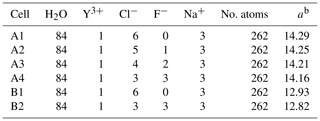a Volume estimated using the empirical equation of state from for 2 molal NaCl solution. b Edge length of the simulation box (Å).

2 Methods

## 2.1 Ab initio molecular dynamics

The AIMD simulation approach is based on a quantum-mechanical description of the electronic structure within the density functional theory (DFT) . Here, we used AIMD simulations with the Car–Parrinello method to model the molecular structure of $\mathrm{Y}-\left(\mathrm{Cl},\mathrm{F}\right)$ complexes in aqueous solutions. We performed simulations with the widely used Car–Parrinello molecular dynamics (CPMD) code . Within the code, the BLYP exchange correlation functional (Becke1988) was employed and the plane-wave expansion of the Kohn–Sham orbitals was truncated at a cutoff energy of 80 Ry. To reduce the computation effort, the core electrons of all atoms in the cubic simulation cell were approximated by Goedecker-type pseudopotentials . To separate the electronic and nuclear motion of the CPMD, a fictive electron mass of 600 $\mathrm{a}.\mathrm{u}.$ with fictitious kinetic energy of 0.24 $\mathrm{a}.\mathrm{u}.$ was used. All results presented here are based on simulations performed with a constant number of atoms N at constant volume V and a temperature T of 800 C (a so-called NVT ensemble) and with a time step of 0.1 fs. The temperature in the simulation was controlled using a Nosé thermostat (Nosé1984; Hoover1985). We chose two different pressure conditions of 1.3 and 4.5 GPa for this study. The volumes of the simulation cell were estimated from the correlation function provided by assuming 2 molal NaCl solution for all cells (see Table 1). Due to this approximation, the pressures listed in Table 1 have to be considered estimates.

The initial atomic configurations were derived from AIMD simulations of pure NaCl solutions (200 H2O and 10 NaCl). This configuration had been equilibrated for a few tens of picoseconds (ps) at 1000 K and a simulation box size of 13.74 Å. The original NaCl solutions were generated in classical molecular dynamics simulations using Matsuoka–Clementi–Yoshimine (MCY) potentials . We substituted one of the sodium atoms by yttrium and decreased the number of water molecules and chlorine atoms stepwise until we reached configuration A1 in Table 1. Figure 1 shows a snapshot of the simulation cell A1 with a [YCl3(H2O)4]aq complex. The chloride ions not initially bonded to the yttrium ion are constrained to remain at larger distance (6–7 Å) from the yttrium ion. All other simulation boxes (see Table 1) were generated from this initial one and equilibrated for several picoseconds.

For the fluoride-bearing cells, we used different cell compositions due to the strong association of hydrogen and fluoride at low pressures. Only initially bonded F ions were included to avoid the formation of hydrofluoric acid by the reaction ${\mathrm{H}}_{\mathrm{2}}\mathrm{O}+{\mathrm{F}}^{-}⇌{\mathrm{OH}}^{-}+\mathrm{HF}$.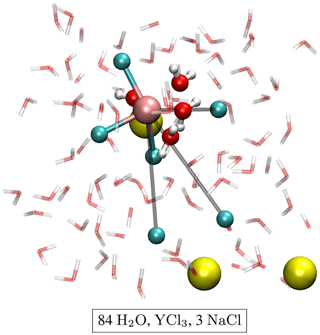Figure 1Snapshot of simulation cell A1 with a [YCl3(H2O)4]aq complex. The water molecules are indicated by red–white bond sticks, sodium by yellow balls and chlorine by cyan balls. The H2O in the first hydration shell of the yttrium atom (copper colored) is presented as red–white balls and sticks. The constraint distances between the yttrium ion and the constraint Cl are colored in gray.

## 2.2 Analysis of interaction distances and coordination number

The average atomic structure of disordered systems such as aqueous solutions is commonly described in terms of partial radial distribution functions gij(r). These functions describe the probability for finding a pair of atoms of elements j and i at a distance r normalized to the particle number density ρN of the fluid:

$\begin{array}{}\text{(1)}& {g}_{ij}\left(r\right)=\frac{\mathrm{1}}{{c}_{\mathrm{i}}{c}_{j}\mathrm{4}\mathit{\pi }{r}^{\mathrm{2}}{\mathit{\rho }}_{N}N}\sum _{a=\mathrm{1}}^{{N}_{\mathrm{i}}}\phantom{\rule{0.33em}{0ex}}\sum _{b=\mathrm{1}}^{{N}_{j}}\mathit{\delta }\left(r-|{\mathbit{R}}_{a}-{\mathbit{R}}_{b}|\right),\end{array}$

where ${c}_{\mathrm{i}}\left(={N}_{\mathrm{i}}/N\right)$ and cj are the concentrations of elements i and j, N is the number of particles in the simulation box, δ(x) the Dirac delta function, and Ra and Rb are the position vectors of particles a and b. In the numerical implementation of Eq. (1), the distance of each particle in respect to all other particles is calculated at every time step. The evolving list of distances is normalized to the number of particles and the volume of the simulation cell.

The positions of the first maximum of gij(r) represent the distances with the highest density of particles around the central position, which are usually interpreted as the nearest-neighbor distances (or bond distances) between elements i and j. The average coordination number of one element is derived from counting the number of neighbors for each atom of this element within a given cutoff distance respecting periodic boundary conditions and averaging over all particles of the same kind and over time. The cutoff distance is taken from the first minimum of the respective gij(r). The association of OH groups with cations is evaluated by considering oxygen atoms coordinated by one hydrogen only. To distinguish pure OH from two H2O sharing one hydrogen, the cutoff between the oxygen and the hydrogen is set to 1.3 Å. Additionally, the distance of the hydrogen within this cutoff distance to the next oxygen is taken as the distinguishing criterion. Only if the oxygen of the next water molecule is located at a distance of >1.6 Å, the OH is counted as hydroxide. This value represents approximately the hydrogen bond distance between OH and H2O . To evaluate the formation of a certain species during a simulation run, only complexes with a constant coordination of chloride and fluoride over at least 3 ps are considered. The average halogen ion hydration number is computed by counting the number of hydrogen oriented towards the ion of the vicinal water molecules.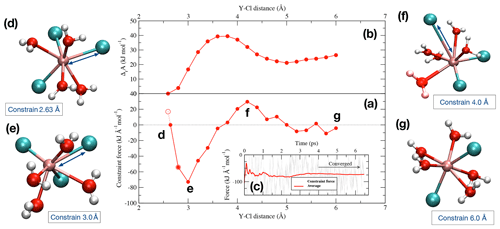Figure 2(a) Potential of mean force of the dissociation reaction of YCl3 to ${\mathrm{YCl}}_{\mathrm{2}}^{+}$ at 1.3 GPa and 800 C over a distance between 2.63 Å and 6.0 Å. The evolution of the Helmholtz free energy is shown in panel (b). In panel (c), an example of the progress of the constraint force with simulation time at a Y–Cl distance of 3.0 Å is shown (stage e). Panels (d)(g) indicate the different stages of the dissociation of the initial complex (see text for details).

## 2.3 Constraint molecular dynamics simulations and thermodynamic integration

A single MD simulation only yields the total internal energy of the system. Thermodynamic integration (TI) is used to derive free energy differences between different states. This approach usually requires a number of intermediate MD simulations along a certain integration variable . Here, we use the constrained molecular dynamics approach and thermodynamic integration in terms of the blue moon sampling . This method has been used already by different groups to investigate the stability of metal complexes in aqueous solutions, not only at ambient pressure and temperature conditions but also at hydrothermal and deep crustal high-density fluid conditions .

Using this method, the Helmholtz free energy difference (ΔrA) of a chemical dissociation reaction is obtained from the average forces F(r) between two atoms under the constraint of keeping their bond distance r constant. ΔrA is derived from the integration of F(r) between two different distances (r1 and r2), which correspond to the associated and the dissociated states of the aqueous complexes :

$\begin{array}{}\text{(2)}& {\mathrm{\Delta }}_{\mathrm{r}}{A}_{\mathrm{1}⟶\mathrm{2}}=-\underset{\mathrm{1}}{\overset{\mathrm{2}}{\int }}〈F\left(r\right)〉\mathrm{d}r.\end{array}$

The formal relation between the Helmholtz free energy and the Gibbs free energy is given by

$\begin{array}{}\text{(3)}& {\mathrm{\Delta }}_{\mathrm{r}}G={\mathrm{\Delta }}_{\mathrm{r}}{A}_{\mathrm{1}⟶\mathrm{2}}+V\underset{\mathrm{1}}{\overset{\mathrm{2}}{\int }}\mathrm{d}P.\end{array}$

V is the volume of the simulation cell. Here, we use an NVT ensemble, and the change in pressure averaged over the whole trajectory is approximately zero (${\int }_{\mathrm{1}}^{\mathrm{2}}\mathrm{d}P=\mathrm{0}$).

To look into the formation of $\mathrm{Y}-\left(\mathrm{Cl},\mathrm{F}\right)$ species in equilibrium reactions, we removed one of the ligands from the Y ion in multiple integration steps by constraining the Y−Cl and Y−F distances. During the integration, the Y−Cl and Y−F distances of the Cl or F ions that are not initially bonded to the yttrium ion are fixed at 6.0 to 7.0 Å to avoid disruptions during the integration. Figure 2 illustrates an example of the dissociation reaction in the simulation box at 1.3 GPa and 800 C:

$\begin{array}{}\text{(R1)}& \left[{\mathrm{YCl}}_{\mathrm{3}}\left({\mathrm{H}}_{\mathrm{2}}\mathrm{O}{\right)}_{\mathrm{4}}{\right]}_{\mathrm{aq}}+{\mathrm{H}}_{\mathrm{2}}\mathrm{O}\to \left[{\mathrm{YCl}}_{\mathrm{2}}\left({\mathrm{H}}_{\mathrm{2}}\mathrm{O}{\right)}_{\mathrm{5}}{\right]}^{+}+{\mathrm{Cl}}^{-}.\end{array}$

The integration starts at the first distance (Fig. 2d), which corresponds approximately to the equilibrium distance of the Y–Cl contact ion pair. This interatomic distance (Fig. 2a) is estimated as the intercept with the zero force line from a linear interpolation between the first and the second integration steps, the first step starting at 2.6 Å for Y–Cl (and at 2.0 Å for Y–F). With increasing displacement of the chloride ion, the constraint force is attractive (Fig. 2e) until a water molecule takes the place of the ion in the first coordination shell around the Y3+ (Fig. 2f). At this point, the force becomes repulsive. By integration over the potential of mean force (PMF) (Fig. 2a), the Helmholtz free energy (ΔrA) difference between the initial complex and the product of the reaction is derived (Fig. 2b). For the Y–Cl complexes, we assume a ligand in a distance of 6.0 Å  as being dissociated, and for Y–F this distance reduces to 5.0 Å (see Fig. S1 in the Supplement ). In Fig. 2g, the dissociation is completed and [YCl2(H2O)5]+ is formed. To estimate the convergence of the constraint force, the standard deviation of the average force is computed. As a convergence criterion, a value of 5 kJ mol−1 over the last 2 ps is taken. This value is also considered as approximate error of the computed reaction free energies. To satisfy this criterion, the constraint AIMD simulations are performed for values between 4.5 and 40 ps.

## 2.4 Thermodynamic approaches

It is textbook knowledge that the formation of monomeric complexes in equilibrium reactions develops in steps . During the formation process, a ligand L is added to the metal cation M. This formation of a MLn complex can be written as a sequence of stepwise reactions:

$\begin{array}{c}M+L\to ML\\ ML+L\to M{L}_{\mathrm{2}}\\ \mathrm{⋮}\\ M{L}_{n-\mathrm{1}}+L\to M{L}_{n},\end{array}$

with the respective logarithmic equilibrium constants:

$\begin{array}{}\text{(4)}& \mathrm{log}\phantom{\rule{0.125em}{0ex}}{K}_{n}=\frac{-{\mathrm{\Delta }}_{\mathrm{r}}G{{}^{\circ }}_{n}}{\mathrm{2.303}RT}.\end{array}$

Having determined equilibrium constants for all of those reactions, the stability constant (also referred to as cumulative stability constant or overall stability constant) βn of species MLn is defined as

$\begin{array}{}\text{(5)}& \mathrm{log}\phantom{\rule{0.125em}{0ex}}{\mathit{\beta }}_{n}=\mathrm{log}\phantom{\rule{0.125em}{0ex}}{K}_{\mathrm{1}}+\mathrm{log}\phantom{\rule{0.125em}{0ex}}{K}_{\mathrm{2}}+\mathrm{log}\phantom{\rule{0.125em}{0ex}}{K}_{\mathrm{3}}\mathrm{\dots }\mathrm{log}\phantom{\rule{0.125em}{0ex}}{K}_{n}.\end{array}$

The standard Gibbs free energy (${\mathrm{\Delta }}_{\mathrm{r}}G{{}^{\circ }}_{\mathrm{i}}$) depends on the reaction Gibbs free energy (ΔrGi) derived from the MD simulation, temperature T, gas constant R, molality of the ions mi and the activity coefficient γi:

$\begin{array}{}\text{(6)}& {\mathrm{\Delta }}_{\mathrm{r}}G{{}^{\circ }}_{\mathrm{i}}={\mathrm{\Delta }}_{\mathrm{r}}{G}_{\mathrm{i}}-R\phantom{\rule{0.125em}{0ex}}T\phantom{\rule{0.33em}{0ex}}\mathrm{ln}\frac{{m}_{M{L}_{\mathrm{i}}}{\mathit{\gamma }}_{M{L}_{\mathrm{i}}}}{{m}_{M{L}_{\mathrm{i}-\mathrm{1}}}{\mathit{\gamma }}_{M{L}_{\mathrm{i}-\mathrm{1}}}\cdot {m}_{L}{\mathit{\gamma }}_{L}}.\end{array}$

The standard state for a solute in aqueous solution is a 1 molal hypothetical solution with properties of an infinitely diluted solution (IUPAC1982). The concentration and behavior of the solutes in the simulation cell (see Table 1) are quite different from this standard state. Therefore, we computed the activity coefficient corresponding to this hypothetical solution using the B-dot model , which is an empirical extension of the Debye–Hückel theory :

$\begin{array}{}\text{(7)}& \mathrm{log}{\mathit{\gamma }}_{\mathrm{i}}=-\frac{{z}_{\mathrm{i}}^{\mathrm{2}}\phantom{\rule{0.125em}{0ex}}{A}_{\mathrm{DH}}\sqrt{I}}{\mathrm{1}+{\mathit{å}}_{\mathrm{i}}\phantom{\rule{0.125em}{0ex}}{B}_{\mathrm{DH}}\sqrt{I}}+\stackrel{\mathrm{˙}}{B}I,\end{array}$

where zi is the charge of ion i, å the mean distance of closest approach between the ions and I the ionic strength:

$\begin{array}{}\text{(8)}& I=\mathrm{0.5}\sum _{i=\mathrm{1}}^{n}{m}_{\mathrm{i}}{z}_{\mathrm{i}}^{\mathrm{2}}.\end{array}$

ADH and BDH are the Debye–Hückel parameters:

$\begin{array}{}\text{(9)}& {A}_{\mathrm{DH}}=\frac{\mathrm{1.8248}\cdot {\mathrm{10}}^{\mathrm{6}}\sqrt{{\mathit{\rho }}_{{\mathrm{H}}_{\mathrm{2}}\mathrm{O}}}}{\left(T\mathit{ϵ}{\right)}^{\frac{\mathrm{3}}{\mathrm{2}}}}\text{(10)}& {B}_{\mathrm{DH}}=\frac{\mathrm{50.292}\sqrt{{\mathit{\rho }}_{{\mathrm{H}}_{\mathrm{2}}\mathrm{O}}}}{\left(T\mathit{ϵ}{\right)}^{\frac{\mathrm{1}}{\mathrm{2}}}}.\end{array}$

They depend on temperature, density (${\mathit{\rho }}_{{\mathrm{H}}_{\mathrm{2}}\mathrm{O}}$) and dielectric constant (ϵ) of the solvent. ϵ is computed using the equation provided by and for pure H2O assuming a fluid density of the simulation. The value of $\stackrel{\mathrm{˙}}{B}$ is calculated by the CHNOSZ software package (Dick2008) applying the extrapolation suggested by . The $\stackrel{\mathrm{˚}}{a}$ parameter for the different complexes and ions (for all $\mathrm{Y}-\mathrm{Cl}/\mathrm{F}$ complexes, a constant value of 4.5 Å is applied) are taken from and Eq. (7) was solved in a Python implementation of the EQBRM program .

Table 2The listed atomic distances and coordination numbers are averaged over the whole-simulation runs for all unbiased simulations. “AIMD time” corresponds to the total simulation time, whereas lifetime (“LT”) refers to persistence of the initial yttrium–halide complex. Under “formed”, the most abundant complexesa of the last 10 ps of the simulation are listed.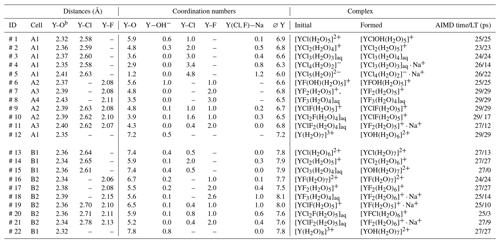a A list of all formed complexes observed for at least 3 ps over the AIMD is given in the Supplement in Table S3. b The decomposed Y−OH2 and $\mathrm{Y}-{\mathrm{OH}}^{-}$ distances are listed in Table S1.

Note that in the simulations we modeled a dissociation reaction but to be in line with the modern nomenclature of aqueous geochemistry all the derived thermodynamic data presented below correspond to the formation reaction. In this study, we investigated two kinds of reactions:

$\begin{array}{}\text{(R2)}& {\mathrm{Y}}^{\mathrm{3}+}+n{\mathrm{Cl}}^{-}\to {\mathrm{YCl}}_{n}^{\mathrm{3}-n}\phantom{\rule{0.125em}{0ex}}\phantom{\rule{0.125em}{0ex}}\left(n=\mathrm{1}-\mathrm{3}\right)\end{array}$

and

$\begin{array}{}\text{(R3)}& {\mathrm{Y}}^{\mathrm{3}+}+n{\mathrm{F}}^{-}\to {\mathrm{YF}}_{n}^{\mathrm{3}-n}\phantom{\rule{0.125em}{0ex}}\phantom{\rule{0.125em}{0ex}}\left(n=\mathrm{1}-\mathrm{3}\right).\end{array}$

In the following, all results referring to one of those reactions are indexed by TI n. Furthermore, for reasons of clarity, we do not include the number of hydration water molecules in the formula of the aqueous complexes in some of the presented figures and tables.

3 Results

## 3.1 Yttrium coordination in high-density aqueous fluid

AIMD simulations were performed for the hydrated Y3+ and for 11 different yttrium–halogen complexes: five YCl${}_{n}^{\mathrm{3}-n}$, n=1–5; three YF${}_{n}^{\mathrm{3}-n}$, n=1, 2, 3; and three mixed $\mathrm{Y}-\left(\mathrm{Cl},\mathrm{F}\right)$ complexes. Simulation conditions and obtained structural data are compiled in Table 2. Moreover, the formed aqueous species are listed in Table 2. Note that the composition of the simulation cells varies slightly as cells A1–A4, B1 and B2 contain different amounts of F and Cl.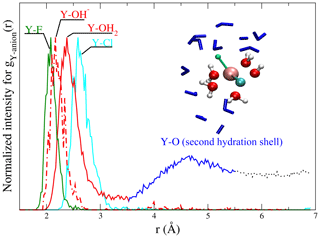Figure 3Radial distribution functions of $\mathrm{Y}-\left(\mathrm{Cl},\mathrm{O},\mathrm{F}\right)$ scaled to the maximum of the gij(r) from run # 9 and 12 together with a snapshot of a [YClF(OH)(H2O)4] complex. In the snapshot (from a run at 1.3 GPa and 800 C), the central yttrium atom is surrounded by chlorine (cyan), fluorine (green), a hydroxyl group and water molecules (red – O and white – H balls). Water molecules of the second shell are shown as blue sticks. This visualization illustrates the relation between gij(r) and the atomic structure of the aqueous species. The colors of the ligands in the snapshot are equivalent to those in the gij(r) functions.

Different partial radial distribution functions for Y–(Cl, F, OH and OH2) are shown in Fig. 3. To facilitate the comparison of the first peaks, the gij(r) values are scaled to equal maximum intensity. The observed sequence of atomic distances between the central metal ion and its ligands holds for all the complexes. The shortest distance is found between Y3+ and F (∼2.1 Å), followed by $\mathrm{Y}-{\mathrm{OH}}^{-}$ (∼2.1–2.2 Å) and Y–OH2 (∼2.3–2.4 Å). The largest distance is observed for Y–Cl pairs (∼2.6–2.8 Å) (for an overview, see Tables 2 and S1). Further, in gY−O(r), a second maximum is observed. It corresponds to the second hydration shell, which is formed around all complexes at all studied PT conditions. For all fluid compositions, association of NaCln (n=1–3) and of Na+ with the yttrium complex is observed. The mean Na–Cl coordination, NaCl species distribution and second hydration shell positions are listed in Table S1.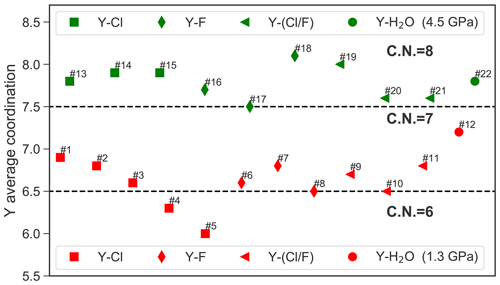Figure 4Average yttrium coordination by chloride, fluoride and oxygen for run # 1–22.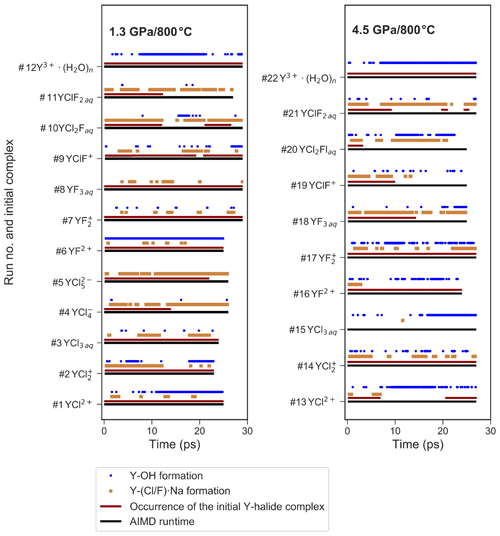Figure 5Presence of selected ion pairs or complexes during the different AIMD simulations. This includes the formation of OH within the first hydration shell of yttrium, the association of sodium with the coordinating halogens and the stability of the initial Y–halide complexes over AIMD time.

At 1.3 GPa, the average Y coordination by O, Cl and/or F is about seven (see Fig. 4) with two exceptions, run # 4 and 5, even if the initial coordination is lower (run # 1–3). In run # 1–3, the initial Y–Cl coordination is retained over the entire AIMD runtime, whereas for # 4 and 5, the 4-fold and 5-fold Cl coordinations do not persist and the time average yttrium coordination is below seven. The initial complex of # 5 seems to be unstable and transforms to the initial complex of # 4 after 26 ps. In # 4, after the 4-fold coordinated chloride complex is dissociated, a total coordination number of seven is reached at the end of the simulation run. Frequently, the formation of OH in the first hydration shell of yttrium is observed. The major hydroxide formation mechanism will be discussed below.

Figure 5 provides an overview over the formation and dissolution of selected structural units in the course of the simulations, i.e., the stability of the initial complexes, Y–hydroxide association and bonding of the coordinating halogen to sodium. All five chloride complexes associate with sodium. The strongest association is observed in # 2–5, where the sodium is connected to one or two chloride ligands of the Y complex. This association increases with the number of halide ligands in these complexes. Furthermore, in # 4 and 5, this association initiates the dissociation of [YCl4(H2O)2] and [YCl5(H2O)]2−. Even larger clusters of sodium, constraint chlorides and the Y–chloride complex appear over timescales of less than 3 ps. Moreover, from # 1–5, the average Y coordination decreases with the increasing number of initial chloride ligands (Fig. 4). The Y–O distances do not change significantly with the increasing number of chloride ligands from # 1 to 4. Only in run # 5 is a significantly longer Y–O distance of 2.41 Å observed. The Y–chloride distances range from 2.58 Å in # 1 to 2.63 Å in # 5.

For pure Y–fluoride complexes under the same conditions (# 6–8), a slight increase of the Y–O distance with increasing number of fluoride ligands from 2.37 Å in [YF(H2O)6]2+ to 2.43 Å in [YF3(H2O)4]aq is observed. In all three runs, the initial complex persists over the whole simulation time. As in the case of Y–chloride solutions, the association of fluoride with sodium is observed but it is less pronounced. In # 6, where only one fluoride is initially bonded to the central ion, OH is formed within the first hydration shell of yttrium. The Y−F distances within the complexes are approximately 0.5 Å shorter than those of the Y−Cl species.

For the $\mathrm{Y}-\left(\mathrm{Cl},\mathrm{F}\right)$ mixed complexes (run # 9–11), only the run starting initially from [YClF(H2O)5]+ does not show the formation of multiple complexes over time. Here, only short separations of the Cl over ∼1ps from the complex occur. In # 10, [YCl2F(H2O)4]aq dissociates to [YClF(H2O)6]+ after 11.5 ps. This complex is present over approximately 10 ps, in conjunction with the formation of [YClFOH(H2O)4]aq, followed by the reassociation of the initial complex. In # 11, starting from [YClF2(H2O)4]aq, the initially bonded chloride is released after ∼12ps and [YF2(H2O)5]+ is formed. The $\mathrm{Y}-\left(\mathrm{Cl},\mathrm{F}\right)$ distances of the mixed complexes are comparable to those of the pure ones.

In run # 12, starting from [Y(H2O)7]3+, hydroxide is formed within the first 8 ps (see Fig. 5), which results in the formation of [YOH(H2O)6]2+ that is present over 14 ps of the AIMD time, followed by a reassociation and a redissociation, which suggests a dynamic change between these two species.

In the high-pressure runs at 4.5 GPa (# 13–22), the average Y coordination is about eight (see Fig. 4). In the case of the Y–chloride complexes, the dissociation of the 1-fold and 3-fold coordinated complexes is observed in run # 13 and 15 (see Fig. 5). Only in run # 14 does the initial Y–chloride complex [YCl2(H2O)5]+ persist over the whole 27 ps trajectory. The higher-coordinated Y–chloride complexes break apart within the equilibration run and the results are not further analyzed. This breakdown is partly driven by the association of the coordinating chloride with sodium. For instance, in run # 13, one sodium chloride unit associates with the Y complex before the chloride dissociates from the Y complex and [NaCl3]2− is formed for ∼3ps (Fig. 6). The resulting [Y(H2O)8]3+ associates with OH shortly afterwards. In all high P runs, the formation of OH by self-dissociation of H2O close to the yttrium ion can be seen as in the low P runs.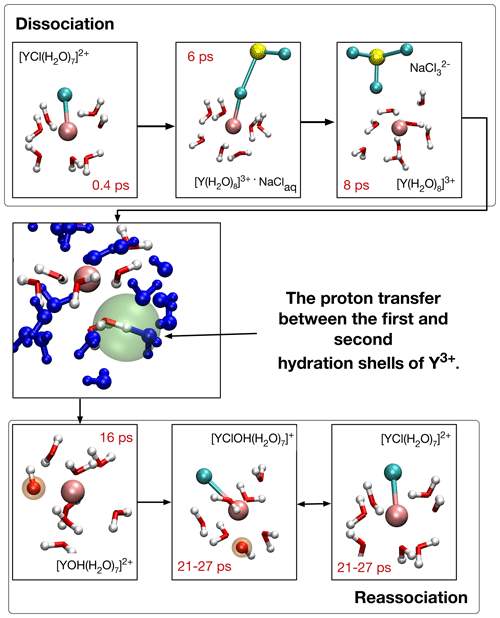Figure 6Formation of [YOH(H2O)7]2+ and reassociation of initial complex [YCl(H2O)7]2+ in run # 13. The blue-colored water molecules are located in the second hydration shell, red–white H2O and OH are bonded in the first coordination shell, and sodium is colored yellow. Chloride ions are in cyan and the Y3+ ion is copper colored. The red numbers indicate the time progress in the AIMD simulation. In the center, the proton transfer state is highlighted with a green sphere.

Figure 6 illustrates the OH formation mechanism as it evolves for Y–chloride and Y–fluoride complexes at low- and high-pressure conditions for the example of [YCl(H2O)7]2+ in run # 13. After the initial complex dissociates within the first 7 ps into [Y(H2O)8]3+, a proton is transferred between one H2O in the first hydration shell and a water molecule of the second shell after additional 2–3 ps. The resulting [YOH(H2O)7]2+ complex is present over 14 ps, followed by reassociation with chloride. The thus-formed [YClOH(H2O)6]+ persists during the remaining simulation time with some interruptions due to short-lived proton transfers.

For the Y–fluoride complexes, under the high-pressure conditions, the 1-fold or 2-fold Y by F coordination persists over the whole-simulation runs (# 16, 17). All complexes show association with one or two sodium ions over several picoseconds but this interaction does not lead to a dissociation of fluoride from yttrium. In # 18, the initially 3-fold coordinated complex dissociates after 14.5 ps and [YF2(H2O)6]+ is formed. None of the mixed $\mathrm{Y}-\left(\mathrm{Cl},\mathrm{F}\right)$ complexes at 4.5 GPa persist over the entire simulation run. In each of those runs, all chloride ions are dissociated from the yttrium after at most 10 ps, and pure Y−F or $\mathrm{Y}-\left(\mathrm{F},\mathrm{OH}\right)$ complexes remain. As in the low-pressure run for the pure hydrated Y3+, a hydroxide ion is observed in the first hydration shell for a significant amount of simulation time.

The nearest Y-(O,Cl,F) distances show only small variations between both pressure conditions, typically in the range of 0.01–0.06 Å for the stable complexes (Table 2). A closer look at the distances between oxygen of the second hydration shell and the yttrium ion (see Y−O(2nd) in Table S1) reveals a continuous increase from the purely hydrated ion with increasing Cl coordination at low pressures from 4.7 to 5.2 Å. In all other cases, these distances are rather similar in a range between 4.3 and 4.6 Å.

Comparing the average halide ion coordination by H2O molecules, differences between purely hydrated halide ions or halide ions associated with the yttrium ion as well as pressure-induced changes are observed (see Table S2). For the chloride ion at 1.3 GPa, the number of hydrating water molecules increases from two to four between ${\mathrm{YCl}}_{\mathrm{2}}^{+}-{\mathrm{YCl}}_{\mathrm{3}\phantom{\rule{0.125em}{0ex}}\mathrm{aq}}$ and dissociated Cl, whereas in the mixed complexes YClF2 aq the chloride hydration number is close to three. For YCl2+, ${\mathrm{YCl}}_{\mathrm{4}}^{-}$ and ${\mathrm{YCl}}_{\mathrm{5}}^{\mathrm{2}-}$, this number lies between two and three. The fluoride ion is coordinated by one water molecule in the Y–fluoride and mixed complexes. At 4.5 GPa, F is hydrated by four H2O and by two when associated with the metal ion, whereas the dissociated Cl coordination increases to five solvent molecules. For those Y–chloride complexes that persist for at least 10 ps in the AIMD run, the chloride ion is coordinated by three water molecules.

To conclude this section, the main findings from the AIMD simulations are summarized. Firstly, the pure Y–chloride complexes YCl2+, ${\mathrm{YCl}}_{\mathrm{2}}^{+}$ and YCl3 aq do not dissociate within the course of the simulation of at least 23 ps at a pressure of 1.3 GPa (ρ=1072kg m−3) but they do at higher pressure (4.5 GPa, 1447 kg m−3) except for YCl${}_{\mathrm{2}}^{+}$. Furthermore, ${\mathrm{YCl}}_{\mathrm{4}}^{-}$ and ${\mathrm{YCl}}_{\mathrm{5}}^{\mathrm{2}-}$ decompose in the unbiased AIMD simulations. Secondly, the pure Y–fluoride complexes persist over the simulation time at 1.3 GPa. It is important to state that at these conditions the formation of hydrofluoric acid is very strong when the fluoride is not associated with the metal ion. At 4.5 GPa, the neutral complex [YF3(H2O)5]aq dissociates and a lower coordinated species forms. Thirdly, OH is formed in the first hydration shell of Y–chloride and Y–fluoride complexes due to the self-dissociation of water. Its abundance increases with decreasing number halide ligands. Furthermore, chloride as well as fluoride form mixed complexes with yttrium and hydroxide at both PT conditions, whereas mixed $\mathrm{Y}-\left(\mathrm{Cl},\mathrm{F}\right)$ complexes are rather unstable. The overall coordination of yttrium changes from ∼7 at 1.3 GPa to ∼8 at 4.5 GPa.

## 3.2 Free energy exploration

Although several complexes are observed in some of the runs described above, it is not feasible to derive the corresponding formation constants of the aqueous species directly from the AIMD simulations. This would require much longer simulation times to ensure the statistical significance of the relative species abundance. On the tens of picoseconds timescale, we did not observe a complete exchange of the halogen ligands of the Y–chloride or Y–fluoride complexes except for run # 15. To overcome this problem, we apply the TI approach within a constraint AIMD simulation to compute the reaction free energies of aqueous complex dissociation. As described in the previous section, the mixed $\mathrm{Y}-\left(\mathrm{Cl},\mathrm{F}\right)$ complexes have a tendency to dissociate already during the conventional AIMD runs, which indicates their low stability. Therefore, no TI runs are performed for those complexes. When the constraint halide ion associates with hydrogen during the constraint MD, the simulation is stopped and the integration step is repeated from a different starting configuration. Thus, we confirm that all results are reproducible within a series of simulations. The derived Helmholtz free energies (ΔrA) of Reactions (R2) and (R3) are listed in Table 3.

Table 3List of the formation Gibbs free energies (kJ mol−1) derived from ab initio thermodynamic integration. The error of the free energies is estimated with 5 kJ mol−1.At 1.3 GPa, in TI-1 starting from [YCl(H2O)6]2+, in nearly all TI steps close to the yttrium OH formed by the hydrolysis of water molecules within the first 2–4 ps. Therefore, the obtained dissociation energy of 36.1 kJ mol−1 does not distinguish between [YCl(H2O)6]2+ and [YOHCl(H2O)5]+ complexes. For TI-2, we observe a similar dissociation energy of 38.8 kJ mol−1 but much fewer hydroxide ions are formed such that in average over all integration steps $\mathrm{Y}-{\mathrm{OH}}^{-}$ appears in only 14 % of the total simulation time. The lowest dissociation energy of the Y–chloride complexes at 1.3 GPa occurs in TI-3 with 26.4 kJ mol−1. In TI-3, only little amounts of OH are formed. While the integration proceeds, the yttrium–oxygen coordination changes (including OH and H2O) for all complexes at a constraint Y−Cl distance between 3.6 and 4.0 Å. The removed chloride as well as the remaining Y–chloride complex associate with sodium during the dissociation process for a few picoseconds. Dissociation energies of the Y–fluoride complexes at 1.3 GPa could not be obtained due to the strong association of H+ and F. The formation of hydrofluoric acid prevents the required long constraint Y–fluoride bond distances for the integration of the PMF.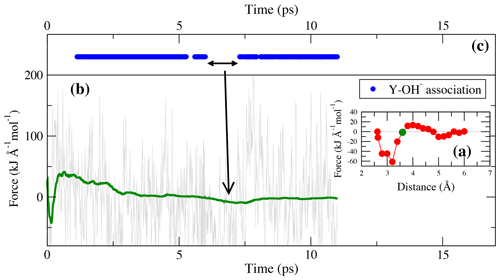Figure 7(a) Potential of mean force of TI-4. The green circle indicates the constraint distance of 3.6 Å for which the force and cumulative mean force is shown in panel (b). Dots in panel (c) indicate the presence of $\mathrm{Y}-{\mathrm{OH}}^{-}$ bonds during the simulation run.

As in the lower-pressure runs, the integration at high pressure does not distinguish between complexes in which OH is present or absent. In TI-4, starting from [YClOH(H2O)6]+ and forming [YOH(H2O)7]2+, a free energy difference of 29.6 kJ mol−1 is obtained. As illustrated in Fig. 7, the reassociation of OH with an excess proton leads to a change of the constraint force due to the decreasing attraction of the metal cation to the constraint chloride ligand. The most frequent $\mathrm{Y}-{\mathrm{OH}}^{-}$ association is observed at constraint Y–Cl distances above 3.0 Å.

The dissociation energies of the Y–chloride complexes significantly decrease between [YCl(H2O)7]2+ and [YCl2(H2O)6]+ at 4.5 GPa. TI-5 yields a low dissociation energy of 8.5 kJ mol−1 where in several of the integration steps the second chloride ligand also dissociates and [YOH(H2O)7]2+ is formed. The Y−Cl dissociation is preceded by an $\mathrm{Y}-{\mathrm{OH}}^{-}$ association and results in the formation of $\left[{\mathrm{YOH}}_{\mathrm{0}-\mathrm{1}}\left({\mathrm{H}}_{\mathrm{2}}\mathrm{O}{\right)}_{\mathrm{6}-\mathrm{7}}{\right]}^{\mathrm{3}-\mathrm{2}+}$. In those cases, the initial complex was reset and the integration step was restarted. For [YCl3(H2O)5]aq, the dissociation energy was not derived because the initial complex dissociated at short Y−Cl constraint distances within the first picosecond of each simulation. Therefore, this complex is not considered as important at such high-pressure conditions. An approach to derive a dissociation energy for such unstable complexes was shown by by constraining the remaining ligands at the equilibrium distance.

Table 4List of the logarithmic stability constants log  β* derived from ab initio thermodynamic integration in comparison to theoretical predictions based on HKF regression.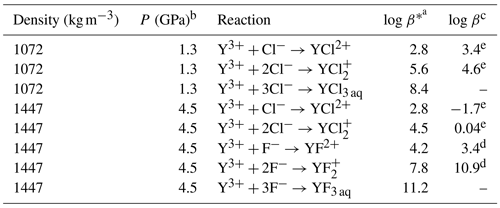a Listed values involve further transition states that cannot be separated during the TI. b Pressure estimates assuming a 2 molal NaCl solution using the empirical equation of state by . c Values computed using the DEW model with HKF parameters reported by d for Y and e for Ho complexes.

In TI-6 to TI-8, the dissociation energies of Y–fluoride complexes following Reaction (R3) are investigated. The equilibrium distance between the yttrium ion and its fluoride ligands is smaller than the Y–Cl bond distance. Due to this shorter distance, the integration range to reach the dissociated state was reduced to 5.0 Å. As shown in Fig. 8 (and Fig. S1), the convergence of the free energy is still reached. In each run of this simulation series, we observe the temporary formation of hydrofluoric acid by the protolysis from H3O+ to one of the constraint Fand, compared to the low-pressure runs, to a lesser extent by the protolysis of H2O.

The dissociation energies (Fig. 8) are quite similar between the Y–fluoride complexes. During the integration, the Y complexes as well as the removing fluoride ions interact with sodium. No self-dissociation of the complexes is observed except for [YF3(H2O)5]aq. In the latter case, at a constant distance of 2.6 Å, one of unconstrained fluorides separates from the initial complex. However, this behavior is not reproducible.

During all integration runs at both pressures, the Y−O coordination number increases in average by one during the transformation from the associated with the dissociated state. For the Y–chloride complexes, the increase in hydration happens at a Y−Cl distance between 3.6 and 4.1 Å, which is in the range of the repulsive part of the constraint force. For the dissociation of Y–fluoride complexes, this distance decreases to 3.4–3.6 Å. A significant influence of the second hydration shell on the constraint force of the reaction coordinate is not observed. All complexes and the removing halide ions interact with sodium. This interaction cannot be quantified by the PMF but the association of the yttrium complex with sodium decreases with the number of halide ligands.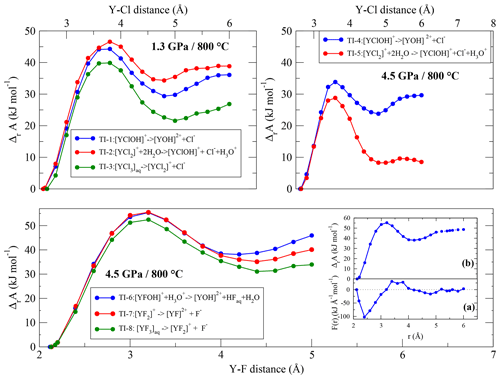Figure 8Evolution of the Helmholtz free energy derived from thermodynamic integration for $\mathrm{Y}-\mathrm{Cl}/\mathrm{F}$ complexes at 800 C and 1.3∕4.5GPa Inset: (a) potential of mean force of the dissociation reaction of [YFOH]+ to ${\mathrm{YOH}}_{\mathrm{2}}^{+}$ for a integration length of 5.0 and 6.0 Å. (b) Resulting Helmholtz free energy along the integration pathway (for higher magnification, see Fig. S1).

## 3.3 Thermodynamic data

Finally, the reaction free energies derived from thermodynamic integration are transformed into standard state properties by applying the activity corrections described in the methods section above. The standard state correction yields ΔrG values that are significantly smaller compared to ΔrG (see Table 3). This treatment does not consider explicitly the formation of HCl and HF as well as the association of yttrium with hydroxide because their formation during TI is not systematic and it is not possible to quantify their contributions to the reaction free energies. As these contributions seem not to be negligible as shown in Fig. 7, the logarithmic stability constants include not only the reactions listed in Table 4 and are therefore indicated with a star (log  β*). Figure 9 shows the ΔrG and log β* for the different species. While pressure does not affect the formation reaction of YCl2+, the stability of ${\mathrm{YCl}}_{\mathrm{2}}^{+}$ decreases substantially with increasing pressure. Comparing the stability constants of chloride and fluoride species at 4.5 GPa, it is found that those of the fluoride species are higher by 1.4 ($\mathrm{log}{\mathit{\beta }}_{\mathrm{1}}^{*}$) to 3.3 ($\mathrm{log}{\mathit{\beta }}_{\mathrm{2}}^{*}$) log units.

4 Discussion

## 4.1 Molecular structure of the aqueous complexes

As mentioned in the introduction, the number of studies focusing on the hydration environment of yttrium or other HREEs in aqueous fluids at high T and P conditions is very limited. The average coordination of seven nearest neighbors that is observed in the simulations at 1.3 GPa fits in the range of experimental results. observed eight to nine nearest neighbors at lower T of 250 C and vapor pressures in highly concentrated chloride solution. But they did not find an association of Y–chloride, whereas reported a strong association with an average coordination of four at 500 C. The present simulations predict that ${\mathrm{YCl}}_{\mathrm{4}}^{-}$ is not stable at high PT conditions. The reason for this could be the too-low average Y coordination in ${\mathrm{YCl}}_{\mathrm{4}}^{-}$ and ${\mathrm{YCl}}_{\mathrm{5}}^{\mathrm{2}-}$. An average coordination of seven as present in the stable Y–chloride complexes of the simulations cannot be achieved in these highly chlorinated species due to steric constraints. The Y–O distances derived from EXAFS spectra by in the range of 2.36–2.39 Å are in good agreement with the atomic distances from the presented simulations, while the conference abstract by does not comprise quantitative data. Experiments and simulations are only partly comparable, as the simulations were performed at higher T (800 C) than the experiments by or .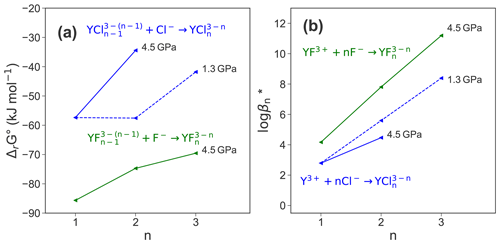Figure 9(a) Reaction Gibbs free energy ΔrG of the different formation reactions; (b) change of the logarithmic stability constant of the different $\mathrm{Y}-\left(\mathrm{Cl},\mathrm{F}\right)$ complexes.

The formation of stable Y−Cl species at 1.3 and 4.5 GPa is consistent with observations by, e.g., , that the mobility of yttrium increases with increasing availability of Cl in aqueous systems. The Y–Cl complexes become less stable with increasing P. The destabilization of metal–halide species with rising pressure is known from a variety of systems (see overview by ). This is commonly explained by the increase of the dielectric constant with increasing density at constant T. The increase of ϵ (ϵ=17.1 at 1072 kg m−3 and ϵ=26.2 at 1447 kg m−3 according to ) leads to a stronger hydration of the metal ion by H2O and the stabilization of charged species. Therefore, YCl3 aq decomposes at 4.5 GPa. The direct competition of both halide ligands in the mixed complexes shows a clear preference of yttrium to form stable bonds with F rather than with Cl. Moreover, the lower reaction Gibbs free energies of the Y–fluoride species in Fig. 9a strongly support this observation.

Figure 10 shows a comparison of the $\mathrm{Y}-{\mathrm{OH}}^{-}$ formation between both pressure conditions in those aqueous complexes that persist over at least 10 ps in the unconstrained AIMD simulations. A higher abundance of hydroxide groups is observed for Y−Cl and Y−F complexes at lower P. Furthermore, the amount of formed OH decreases with increasing number of ligands and is particularly high for the purely hydrated Y3+ at both pressures. This observation cannot be explained by the increased self-dissociation of water, which increases with pressure (see, e.g., ). According to , the OH molality is in the range of 10−6 to 10−5 under the investigated PT conditions. Therefore, the hydroxide formation in the simulation must be driven by charge compensation in the absence of other ligands around the yttrium. The low abundance of $\mathrm{Y}-{\mathrm{OH}}^{-}$ in the neutral species (e.g., YF3 aq) supports this interpretation. It has to be stressed that the initial simulation cells do not contain excess hydroxide ions. Therefore, the $\mathrm{Y}-{\mathrm{OH}}^{-}$ association is expected to be much higher if the OH concentration increases. But it has to be underlined that the values in Fig. 10 are rather imprecise because an equilibrium distribution of Y–hydroxide bonds cannot be achieved in the rather short simulation time.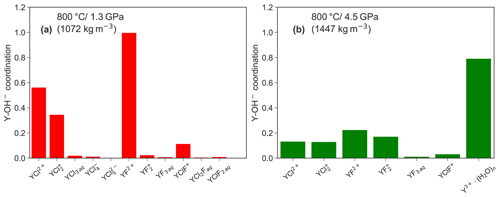Figure 10Comparison of the average $\mathrm{Y}-{\mathrm{OH}}^{-}$ coordination number between the different complexes that exist for at least over 10 ps in the unconstrained AIMD at 1.3 (a) and 4.5 GPa (b).

The association of yttrium with hydroxide as observed in the simulations was also noticed in high PT solubility experiments by in NaCl brines but not in the H2O−NaF system . The authors explained the association by a geometrical effect. The smaller HREEs (in comparison to a LREE) have less attraction to a large chloride ion due to the so-called “steric hindrance”, as discussed by . However, in the case of the Y–fluoride complexes, especially for YF2+, the OH formation is also controlled by the protolysis of H2O close to the metal ion. Therefore, the geometrical explanation does not hold to explain the Y–hydroxide bonding. The fact that this process was not detected in the experiments by might be caused by the high fluoride content in the experiments. The majority of ${\mathrm{YF}}_{\mathrm{2}}^{+}$ in the experiments underlines this conclusion. The fact that the formation of $\mathrm{Y}-\left(\mathrm{F}/\mathrm{OH}\right)$ species was not detected in solubility experiments by up to 250 C indicates that the protolysis of vicinal water by yttrium is a high temperature process. Protolysis at high temperatures was also reported by for Ti4+ and might be a general property of high-field-strength elements.

Entropy is an additional driving force for the ion association due to changes in the local solvent structure near the critical point and above as discussed, e.g., by and based on AIMD simulations. The dominant effect arises from the translational entropy through hydration changes of the ions during the aqueous reaction. Such a concept was already discussed by . They proposed that the change in the electrostriction volume of the solvent controls the association of aqueous species, e.g., NH3 aq, HClaq and NaClaq. In the present simulation study, a relation between the change of hydration of chloride and the stability of certain complexes is observed at 1.3 GPa. For example, the formation of ${\mathrm{YCl}}_{\mathrm{2}}^{+}$ and YCl3 aq (TI-2, TI-3) releases two inner-sphere solvation water molecules because the hydration of Cl decreases from four to two. This may explain the very similar reaction Gibbs energy of 38.8 kJ mol−1 in TI-2 compared to TI-1 (36.1 kJ mol−1), where only one H2O is released (besides the effect of OH formation). Normally, one would expect a decrease in released reaction free energy with increasing number of ligands. Due to the spontaneous formation of HF, the number of H2O in the hydration shell of an Fion cannot be derived directly from the 1.3 GPa simulations. At 4.5 GPa, two hydration H2O are exchanged for Fand Cl during the dissociation of the initial complexes. This convergence of the hydration change for the halogens supports the assumptions by and that the entropic effect decreases with increasing density at constant temperature (≥200C).

## 4.2 Comparison of the thermodynamic data with HKF regression

Experimental high P and high T thermodynamic properties of Y–chloride and Y–fluoride species are not available to compare and evaluate the present simulation results. Therefore, the Deep Earth Water (DEW) model is used to compute stability constants of YF2+ and ${\mathrm{YF}}_{\mathrm{2}}^{+}$ derived from solubility experiments up to 250 C by using a HKF regression to 800 C and a fluid density equal to that of the simulations. There are no stability data of Y–chloride complexes available but due to the similarities of yttrium– and holmium (Ho)–chloride complexes at room temperature , the behavior of Y–chloride complexes is assumed to be similar to Ho–chloride complexes . The Ho–chloride HKF parameters are taken from , . In addition, $\mathrm{Y}/\mathrm{Ho}-{\mathrm{OH}}^{-}$ stabilities are derived from data of , . The results are shown in Fig. 11.

Comparing the results in Fig. 11a, it can be observed that the stabilities of YCl2+ and ${\mathrm{YCl}}_{\mathrm{2}}^{+}$ are similar to those of the Ho–chloride species within approximately one logarithmic unit. For β3 (Y−Cl), Y and Ho show opposite behavior. In this case, an increase in the stability of YCl3 aq is observed, whereas the Ho β3 (Ho−Cl) decreases . do not report any β3 (Cl) values. For Y–hydroxide and Ho–hydroxide species, the stabilities are in the range of YCl2+. At higher density (Fig. 11b), the HKF model yields significantly lower stability constants of Ho–/Y–chloride species compared to the AIMD for YCl2+ and ${\mathrm{YCl}}_{\mathrm{2}}^{+}$. The formation of $\mathrm{Y}-{\mathrm{OH}}^{-}$ in the AIMD runs suggests that $\mathrm{Y}-{\mathrm{OH}}^{-}$ association may occur in high-density brines.

Due to the lack of AIMD log βn (Y−F) data, Fig. 11c only shows values derived from the HKF parameters. Here, the Y–fluoride species have the highest stability compared to Ho–fluoride , Y–hydroxide and Ho–hydroxide species. It should be mentioned here that the model from is suspected to overestimate the HREE-F stability . In Fig. 11d, it is shown that for the higher fluid density the ${\mathit{\beta }}_{\mathrm{1}}^{*}$ (Y−F) values from the simulations are consistent with regression based on experimental data within one log unit. The formation constant of ${\mathrm{YF}}_{\mathrm{2}}^{-}$ from is higher compared to the AIMD results. On the other hand, the Ho–fluoride complexes and the Y–/Ho–hydroxide complexes have a much lower stability. Those differences indicate a different behavior of the geochemical twins (Y and Ho) in fluoride-rich environments, which could explain the fractionation of these elements even at high PT conditions as it was observed, e.g., in hydrothermal systems and discussed by . Furthermore, the comparable stability of both Y–chloride and Ho–chloride complexes confirms the comparable geochemical behavior of the ions in chloride-rich solutions as assumed by .

In general, at lower fluid densities, we note similar stabilities of Y– and Ho–chloride complexes. The strong divergence for the neutral complexes might be explained by the origin of the HKF parameters. The data from were derived from thermodynamic predictions based on measurements at 25 C and 1 bar where only a very small amount of a neutral species is formed and the uncertainty of the extrapolation is large. This interpretation is supported by reported experiments of and , who do not observe the formation of neutral complexes up to 250 C. Only in situ measurements by show higher Cl coordination of yttrium, whereas for Ho no data are available.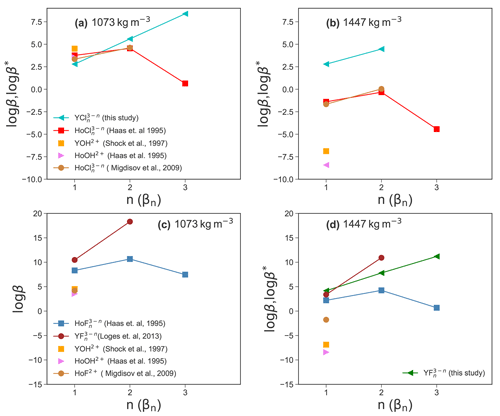Figure 11Comparison of the aqueous species stability derived from the AIMD simulation and HKF regression using the DEW model at 800 C and a fluid density of 1072 and 1447 kg m−3.

## 4.3 The role of chloride and fluoride for the mobilization of Y3+ under subduction-zone conditions

Stable Y–chloride and Y–fluoride complexes are found over the investigated PT range. Fluoride-bearing species are predominant in chloride- and fluoride-rich metamorphic fluids. This outcome is consistent with a variety of studies from the field (see, e.g., ) as well as from experiments . Figure 12a–c show the distribution of the Y–fluoride and Y–chloride complexes as a function of the dissolved salt concentration. In Fig. 12d, the speciation change as function of the Fconcentration is shown. Besides the presented thermodynamic data from this study, competing aqueous reactions as the formation of HClaq, NaClaq, NaFaq, HFaq and NaOHaq as expected in high-grade metamorphic fluids and observed in the simulations are included. Possible complexation with silica components due to the lack of thermodynamic data as well as mineral reactions are not included. The neutral pH∕pOH is fixed by the ion product of water . The thermodynamic properties of the competing aqueous species at high PT conditions are computed using the HKF model parameters reported by , , and using the DEW model . For this simple model, a Y concentration of 23 ppm in solution is assumed. This amount corresponds to measurements in subducted material such as mid-ocean-ridge basalt and is in the range of Y solubility in Cl-bearing brines reported by .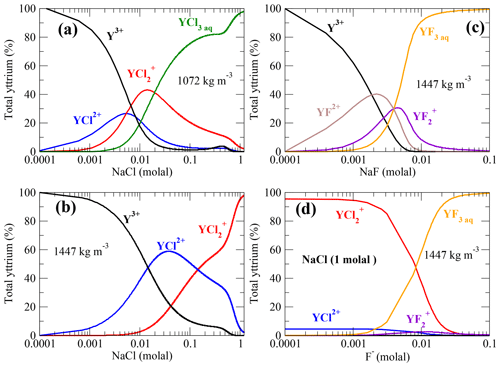Figure 12Y–chloride and Y–fluoride species distribution computed from the AIMD data assuming 23 ppm dissolved yttrium in aqueous solution at 800 C. (a, b) Y–chloride species distribution versus the logarithmic sodium chloride concentration in low- and high-density fluid; (c) Y–fluoride species versus the amount of dissolved NaF and (d) $\mathrm{Y}-\mathrm{Cl}/\mathrm{F}$ species in a 1 molal NaCl solution with increasing F concentration. The pH of the solutions is changing in a range of ±1.7 over the concentration ranges.

Comparing the Y–chloride speciation in Fig. 12a–b, it can be noted that yttrium is mainly associated with Cl at an NaCl concentration of ∼0.005molal in the lower-density fluid, whereas under higher-density conditions this state is reached at ∼0.01molal NaCl in solution. For a fluid density of 1072 kg m−3, YCl3 is the main species above an NaCl concentration of 0.01 molal. Under high-density conditions, ${\mathrm{YCl}}_{\mathrm{2}}^{+}$ is the major species at a higher NaCl concentration of 0.14 molal. This shows that rather small amounts of dissolved NaCl are needed to form Y–chloride species under subduction-zone PT conditions if the yttrium is in solution. The required amounts of chloride presumably occur in subduction fluids as demonstrated in a variety of studies . However, it has been discussed by that fluoride aqueous complexes are much more capable to mobilize significant amounts of yttrium. As shown in Fig. 12c for a fluid-bearing Y+NaF (in the absence of other ligands) at an NaF concentration of about 0.0014 molal, Y–fluoride complexes are the majority species. Deep aqueous fluids in the Earth's crust are presumed to be mixtures of H2O plus salt (mainly NaCl, CaCl2 and KCl) (Manning2018). Therefore, in Fig. 12d, the speciation in a 1 molal NaCl brine with increasing amount of fluoride in the solution at a density of 1447 kg m−3 is computed. Here, ${\mathrm{YCl}}_{\mathrm{2}}^{+}$ and YF3aq are the dominant species. Below a F concentration of approximately 0.01 molal, Y–chloride complexes are formed, and above this concentration, Y–fluoride becomes more important, at least in high-density brines. At lower densities, the formation of Y–fluoride evolves at lower fluoride concentration due to the strong increase of complex stability as shown in Fig. 11c.

Estimates of the fluoride content in aqueous phases evolving in subducting slabs are given in the range of ∼0.003–0.18 molal derived from analysis of melt inclusions and metamorphic rocks , thermodynamic modeling and the fugacity ratio ${f}_{\mathrm{HF}}/{f}_{{\mathrm{H}}_{\mathrm{2}}\mathrm{O}}$ based on partitioning data between mineral and fluid . The simple model outlined above shows that only a low fluoride concentration in the metamorphic aqueous fluids is needed to change the yttrium speciation and therefore its mobility. This outcome is in line with recent thermodynamic modeling by that shows that a small amount of dissolved F (tens of ppm) in ore-forming solutions in equilibrium with a granite assemblage is able to mobilize significant amount of REEs. But the high immobility of yttrium in crustal as well as subduction-zone metasomatism (Ague2017) might reflect a low fluorine activity in the majority of metasomatic fluids due to formation of fluoride species, e.g., HFaq, ${\mathrm{HF}}_{\mathrm{2}}^{-}$, ${\mathrm{SiF}}_{\mathrm{6}}^{\mathrm{2}-}$ or Si(OH)2F2 aq. Therefore, the included thermodynamic properties of HF from might underestimate its stability. A very low solubility of yttrium-bearing minerals as suggested by and the retrograde solubility of REE phosphates could play an important role in the fixation of yttrium and other HREEs.

At high densities, the stability of Y–hydroxide complexes might be higher than the HKF regression indicates. As shown by , at room temperature, the $\mathrm{Y}-{\mathrm{OH}}^{-}$ complexation is sensitive to pH changes and could evolve under more alkaline conditions as presumed for deep metasomatism . But with the applied methods in this study, no further statement can be made due to the limitations of the PMF thermodynamic integration approach to extract reaction free energies for all relevant individual reactions including different intermediate states and dynamic changes such as proton transfer. To overcome this problem, multiple constraints could be applied as suggested by but this would lead to even longer simulation times and might require more than the available computation resources for reaching sufficient convergence of structures and energies . Therefore, other free energy sampling methods could be promising alternatives, e.g., the metadynamics approach . This method has been successfully applied to compute acid constant (pKa) in combination with quantum mechanical molecular dynamics (e.g., ) and to find new reaction pathways (see ). To probe aqueous systems or mineral–fluid interactions of more realistic size and composition, classical molecular dynamics simulations in combination with force field interaction potentials or/and machine learning potentials certainly have potential to provide significant progress in this field.

5 Conclusions

The results of the ab initio molecular dynamics simulations provide new insight into the Y−Cl, Y−F and $\mathrm{Y}-{\mathrm{OH}}^{-}$ complexation in highly saline solutions, as they occur in geological settings, e.g., of subduction zones. Firstly, Y–chloride aqueous complexes are observed at 800 C and 1.3 or 4.5 GPa where YCl3 and ${\mathrm{YCl}}_{\mathrm{2}}^{+}$ are the major species. Moreover, the destabilization of ${\mathrm{YCl}}_{\mathrm{4}}^{-}$ and ${\mathrm{YCl}}_{\mathrm{5}}^{\mathrm{2}-}$ indicates that there are no other Y–chloride species that have to be considered at least in high-grade metamorphic processes.

The extracted thermodynamic properties of Y–chloride species presented in this study are the first published data set to our knowledge. The stability of Ho–chloride complexes derived from thermodynamic calculations based on an HKF regression at a solution density of 1073 kg m−3 suggests that Y and Ho behave very similar in Cl rich solutions but with increasing solution density Y–chloride complexes are more favorable than Ho–chloride complexes. On the contrary, Y shows a much stronger association with fluoride compared to Ho, which could explain their different behavior in F-rich aqueous environments. A different association behavior of both elements with respect to OH would have an even higher impact on the Y∕Ho fractionation because mineral solubilities and mineral surface adsorption are much more controlled by the pH and pOH values than the halide content of the aqueous fluid.

The formation of Y–fluoride complexes in high-density aqueous fluids happens even at very low Fconcentrations and should lead to a high mobility of Y (HREE) as observed in natural samples. Only in very fluorine-rich environments (e.g., ) significant amounts of HREEs are mobilized. This finding may indicate that the fluoride activity in the majority of metamorphic aqueous fluids is rather low. The reason for that could be the high affinity of fluorine to silicate , which is one of the main components of aqueous phases in metamorphic processes . In high-grade metamorphic fluids, it is also necessary to consider that more cations are competing for fluoride to form, e.g., MgF+ and CaF+. This leads to a decrease of the F+ activity as well.

As discussed by Ague (2017), the HREE mass change by fluid–rock interaction is much more determined by the mineral assemblages and phosphate mobility and therefore the halide content of the fluid phase might not be the only controlling factor for the HREE mobility. Nevertheless, the thermodynamic data reported here are consistent with the results of the HKF regression. Furthermore, the stability constants are affected by the formation of hydroxide mixed complexes and HCl∕HF formation during the thermodynamic integration. Therefore, the presented thermodynamic quantities can only be considered as semi-quantitative. Furthermore, it must be emphasized that the applied activity correction could be a source of huge uncertainty. As demonstrated by , the Ewald summation, that is used to build up the periodic boundary condition within the simulation, disrupts the solvation free energy of highly charged ions. But this kind of perturbation is not accounted in the Debye–Hückel approach. Therefore, a more systematic evaluation of the impact of artificial periodic electrostatics and neutralizing background charge on the computed thermodynamic properties derived from AIMD simulations especially at high temperatures is required in future studies.

Code and data availability
Code and data availability.

All simulations were performed using the CPMD code that is distributed free of charge to non-profit organizations under the CPMD Free Licence (https://www.cpmd.org, last access: 27 April 2020). The molecular structures were visualized using the VMD code (; https://www.ks.uiuc.edu/Research/vmd/, last access: 27 April 2020), which is distributed under an Open Source Licence.

Supplement
Supplement.

Author contributions
Author contributions.

JS and SJ designed the study. JS performed the numerical simulations and SJ supervised the analysis and interpretation of the results. Both authors discussed the results of the study and wrote the manuscript.

Competing interests
Competing interests.

The authors declare that they have no conflict of interest.

Special issue statement
Special issue statement.

This article is part of the special issue “Exploring new frontiers in fluids processes in subduction zones”. It is a result of the EGU Galileo conference “Exploring new frontiers in fluids processes in subduction zones”, Leibnitz, Austria, 24–29 June 2018.

Acknowledgements
Acknowledgements.

This study was supported by the Deutsche Forschungsgemeinschaft in the framework of project JA 1469/10-1. The authors gratefully acknowledge the Gauss Centre for Supercomputing e.V. (https://www.gauss-centre.eu, last access: 27 April 2020) for funding this project (CHPO15) by providing computing time through the John von Neumann Institute for Computing (NIC) on the JUWELS supercomputer and JURECA at Jülich Supercomputing Centre (JSC). We appreciate helpful discussions with Thomas Wagner. Yuan Mei and an anonymous reviewer are thanked for their very useful comments and suggestions to improve the manuscript.

Financial support
Financial support.

This research has been supported by the DFG (grant no. JA 1469/10-1) and the Gauss Center for Supercomputing (grant no. CHPO15).

Review statement
Review statement.

This paper was edited by Simone Tumiati and reviewed by Yuan Mei and one anonymous referee.

References

Ague, J. J.: Element mobility during regional metamorphism in crustal and subduction zone environments with a focus on the rare earth elements (REE), Am. Mineral., 102, 1796–1821, https://doi.org/10.2138/am-2017-6130, 2017. a, b, c, d

Alt, J. C., Shanks, W. C., and Jackson, M. C.: Cycling of sulfur in subduction zones: The geochemistry of sulfur in the Mariana Island Arc and back-arc trough, Earth Planet. Sc. Lett., 119, 477–494, https://doi.org/10.1016/0012-821X(93)90057-G, 1993. a

Anderson, G.: Thermodynamics of Natural Systems, Cambridge University Press, Cambridge, 2 Edn., 198–233, 2009. a

Anderson, G. M. and Crerar, D. A.: Thermodynamics in Geochemistry: The Equilibrium Model, Oxford University Press, New York, 1st Edn., 555 pp., 1993. a

Aranovich, L. and Safonov, O.: Halogens in High-Grade Metamorphism, in: The Role of Halogens in Terrestrial and Extraterrestrial Geochemical Processes, edited by: Harlov, D. E. and Aranovich, L., Springer International Publishing, Cham, 713–757, https://doi.org/10.1007/978-3-319-61667-4_11, 2018. a, b, c

Atkins, P. and De Paula, J.: Physical Chemistry, Oxford University Press, 6th Edn., 158 pp., 2006. a

Bali, E., Keppler, H., and Audetat, A.: The mobility of W and Mo in subduction zone fluids and the Mo–W–Th–U systematics of island arc magmas, Earth Planet. Sc. Lett., 351/352, 195–207, https://doi.org/10.1016/j.epsl.2012.07.032, 2012. a

Barnes, J. D., Manning, C. E., Scambelluri, M., and Selverstone, J.: The Behavior of Halogens During Subduction-Zone Processes, in: The Role of Halogens in Terrestrial and Extraterrestrial Geochemical Processes, edited by: Harlov, D. E. and Aranovich, L., Springer International Publishing, Cham, 545–590, https://doi.org/10.1007/978-3-319-61667-4_8, 2018. a

Bau, M. and Dulski, P.: Comparative study of yttrium and rare-earth element behaviours in fluorine-rich hydrothermal fluids, Contr. Mineral. Petrol., 119, 213–223, 1995. a

Becke, A. D.: Density-functional exchange-energy approximation with correct asymptotic behavior, Phys. Rev. A, 38, 3098–3100, https://doi.org/10.1103/PhysRevA.38.3098, 1988. a

Behler, J. and Parrinello, M.: Generalized Neural-Network Representation of High-Dimensional Potential-Energy Surfaces, Phys. Rev. Lett., 98, 146401, https://doi.org/10.1103/PhysRevLett.98.146401, 2007. a

Brown, P. L. and Ekberg, C. (Eds.): Alkaline Earth Metals, Wiley-VCH Verlag GmbH & Co. KGaA, 1–9, 2016. a

Bühl, M. and Golubnychiy, V.: Binding of Pertechnetate to Uranyl(VI) in Aqueous Solution, A Density Functional Theory Molecular Dynamics Study, Inorg. Chem., 46, 8129–8131, https://doi.org/10.1021/ic701431u, 2007. a

Bühl, M. and Grenthe, I.: Binding modes of oxalate in UO2(oxalate) in aqueous solution studied with first-principles molecular dynamics simulations, Implications for the chelate effect, Dalton Trans., 40, 11192–11199, https://doi.org/10.1039/C1DT10796H, 2011. a

Car, R. and Parrinello, M.: Unified Approach for Molecular Dynamics and Density-Functional Theory, Phys. Rev. Lett., 55, 2471–2474, https://doi.org/10.1103/PhysRevLett.55.2471, 1985. a

Cetiner, Z. S., Wood, S. A., and Gammons, C. H.: The aqueous geochemistry of the rare earth elements, Part XIV, The solubility of rare earth element phosphates from 23 to 150 C, Chem. Geol., 217, 147–169, https://doi.org/10.1016/j.chemgeo.2005.01.001, 2005. a

Cheng, B., Engel, E. A., Behler, J., Dellago, C., and Ceriotti, M.: Ab initio thermodynamics of liquid and solid water, P. Natl. Acad. Sci. USA, 116, 1110–1115, https://doi.org/10.1073/pnas.1815117116, 2019. a

Ciccotti, G., Kapral, R., and Vanden-Eijnden, E.: Blue moon sampling, vectorial reaction coordinates, and unbiased constrained dynamics, Chem. Phys Chem., 6, 1809–1814, https://doi.org/10.1002/cphc.200400669, 2005. a

CPMD: Copyright IBM Corp 1990–2018, Copyright MPI für Festkörperforschung Stuttgart 1997–2001, available at: http://www.cpmd.org/ (last access: 27 April 2020), 1990. a, b

Dalou, C., Mysen, B. O., and Foustoukos, D.: In-situ measurements of fluorine and chlorine speciation and partitioning between melts and aqueous fluids in the ${\mathrm{Na}}_{\mathrm{2}}\mathrm{O}-{\mathrm{Al}}_{\mathrm{2}}{\mathrm{O}}_{\mathrm{3}}-{\mathrm{SiO}}_{\mathrm{2}}-{\mathrm{H}}_{\mathrm{2}}$ O system, Am. Mineral., 100, 47–58, https://doi.org/10.2138/am-2015-4859, 2015. a

Dick, J. M.: Calculation of the relative metastabilities of proteins using the CHNOSZ software package, Geochem. T., 9, 10, https://doi.org/10.1186/1467-4866-9-10, 2008. a

Dolejš, D.: Thermodynamics of Aqueous Species at High Temperatures and Pressures: Equations of State and Transport Theory, Rev. Mineral. Geochem., 76, 35–79, https://doi.org/10.2138/rmg.2013.76.3, 2013. a

Dolejš, D. and Zajacz, Z.: Halogens in Silicic Magmas and Their Hydrothermal Systems, in: The Role of Halogens in Terrestrial and Extraterrestrial Geochemical Processes, edited by: Harlov, D. E. and Aranovich, L., Springer International Publishing, Cham, 431–543, https://doi.org/10.1007/978-3-319-61667-4_7, 2018. a

Galvez, M. E., Connolly, J. A. D., and Manning, C. E.: Implications for metal and volatile cycles from the pH of subduction zone fluids, Nature, 539, 420–424, https://doi.org/10.1038/nature20103, 2016. a, b

Goedecker, S., Teter, M., and Hutter, J.: Separable dual-space Gaussian pseudopotentials, Phys. Rev. B, 54, 1703–1710, https://doi.org/10.1103/PhysRevB.54.1703, 1996. a

Goncharov, A. F., Goldman, N., Fried, L. E., Crowhurst, J. C., Kuo, I.-F. W., Mundy, C. J., and Zaug, J. M.: Dynamic Ionization of Water under Extreme Conditions, Phys. Rev. Lett., 94, 125508, https://doi.org/10.1103/PhysRevLett.94.125508, 2005. a

Graupner, T., Kempe, U., Dombon, E., Pätzold, O., Leeder, O., and Spooner, E. T. C.: Fluid regime and ore formation in the tungsten(-yttrium) deposits of Kyzyltau (Mongolian Altai): evidence for fluid variability in tungsten-tin ore systems, Chem. Geol., 154, 21–58, https://doi.org/10.1016/S0009-2541(98)00123-5, 1999. a

Haas, J. R., Shock, E. L., and Sassani, D. C.: Rare earth elements in hydrothermal systems: Estimates of standard partial molal thermodynamic properties of aqueous complexes of the rare earth elements at high pressures and temperatures, Geochim. Cosmochim. Ac., 59, 4329–4350, https://doi.org/10.1016/0016-7037(95)00314-P, 1995. a, b, c, d, e, f, g, h, i

Harlov, D. E., Johansson, L., Van Den Kerkhof, A., and Förster, H.-J.: The Role of Advective Fluid Flow and Diffusion during Localized, Solid-State Dehydration: Söndrum Stenhuggeriet, Halmstad, SW Sweden, J. Petrol., 47, 3–33, https://doi.org/10.1093/petrology/egi062, 2006. a, b

Hartwigsen, C., Goedecker, S., and Hutter, J.: Relativistic separable dual-space Gaussian pseudopotentials from H to Rn, Phys. Rev. B, 58, 3641–3662, https://doi.org/10.1103/PhysRevB.58.3641, 1998. a

Helgeson, H. C.: Thermodynamics of hydrothermal systems at elevated temperatures and pressures, Am. J. Sci., 267, 729–804, https://doi.org/10.2475/ajs.267.7.729, 1969. a

Helgeson, H. C., Kirkham, D. H., and Flowers, G. C.: Theoretical prediction of the thermodynamic behavior of aqueous electrolytes by high pressures and temperatures; IV, Calculation of activity coefficients, osmotic coefficients, and apparent molal and standard and relative partial molal properties to 600 C and 5 kb, Am. J. Sci., 281, 1249–1516, https://doi.org/10.2475/ajs.281.10.1249, 1981. a, b

Hermann, J., Spandler, C., Hack, A., and Korsakov, A. V.: Aqueous fluids and hydrous melts in high-pressure and ultra-high pressure rocks: Implications for element transfer in subduction zones, Lithos, 92, 399–417, https://doi.org/10.1016/j.lithos.2006.03.055, 2006. a

Hermann, J., Zheng, Y.-F., and Rubatto, D.: Deep Fluids in Subducted Continental Crust, Elements, 9, 281–287, https://doi.org/10.2113/gselements.9.4.281, 2013. a

Hetherington, C. J., Harlov, D. E., and Buadzyń, B.: Experimental metasomatism of monazite and xenotime: mineral stability, REE mobility and fluid composition, Miner. Petrol., 99, 165–184, https://doi.org/10.1007/s00710-010-0110-1, 2010. a

Hohenberg, P. and Kohn, W.: Inhomogeneous Electron Gas, Phys. Rev., 136, B864–B871, https://doi.org/10.1103/PhysRev.136.B864, 1964. a

Hole, M. J., Trewin, N. H., and Still, J.: Mobility of the high field strength, rare earth elements and yttrium during late diagenesis, J. Geol. Soc., 149, 689–692, https://doi.org/10.1144/gsjgs.149.5.0689, 1992. a

Hoover, W. G.: Canonical dynamics: Equilibrium phase-space distributions, Phys. Rev. A, 31, 1695–1697, https://doi.org/10.1103/PhysRevA.31.1695, 1985. a

Hückel, E. and Debye, P.: The theory of electrolytes: I. lowering of freezing point and related phenomena, Phys. Z., 24, 185–206, 1923. a

Hughes, L., Burgess, R., Chavrit, D., Pawley, A., Tartèse, R., Droop, G., Ballentine, C. J., and Lyon, I.: Halogen behaviour in subduction zones: Eclogite facies rocks from the Western and Central Alps, Geochim. Cosmochim. Ac., 243, 1–23, https://doi.org/10.1016/j.gca.2018.09.024, 2018. a

Humphrey, W., Dalke, A., and Schulten, K.: VMD – Visual Molecular Dynamics, J. Molec. Graph., 14, 33–38, 1996. a

Hünenberger, P. H. and McCammon, J. A.: Ewald artifacts in computer simulations of ionic solvation and ion-ion interaction: A continuum electrostatics study, J. Chem. Phys., 110, 1856–1872, https://doi.org/10.1063/1.477873, 1999. a

Ikeda, T., Hirata, M., and Kimura, T.: Hydration structure of Y3+ and La3+ compared: an application of metadynamics, J. Chem. Phys., 122, 244507, https://doi.org/10.1063/1.1940029, 2005a. a

Ikeda, T., Hirata, M., and Kimura, T.: Hydration of Y3+ ion: a Car-Parrinello molecular dynamics study, J. Chem. Phys., 122, 024510, https://doi.org/10.1063/1.1832594, 2005b. a, b

IUPAC: A report of IUPAC commission 1.2 on thermodynamics notation for states and processes, significance of the word “standard” in chemical thermodynamics, and remarks on commonly tabulated forms of thermodynamic functions, Tech. Rep. 9, 1982. a

Ivanov, I., Chen, B., Raugei, S., and Klein, M. L.: Relative pKa Values from First-Principles Molecular Dynamics: The Case of Histidine Deprotonation, J. Phys. Chem. B, 110, 6365–6371, https://doi.org/10.1021/jp056750i, 2006. a

Johansson, G. and Wakita, H.: X-ray investigation of the coordination and complex formation of lanthanoid ions in aqueous perchlorate and selenate solutions, Inorg. Chem., 24, 3047–3052, https://doi.org/10.1021/ic00213a035, 1985. a

John, T., Klemd, R., Gao, J., and Garbe-Schönberg, C.-D.: Trace-element mobilization in slabs due to non steady-state fluid-rock interaction: Constraints from an eclogite-facies transport vein in blueschist (Tianshan, China), Lithos, 103, 1–24, https://doi.org/10.1016/j.lithos.2007.09.005, 2008. a

Keppler, H.: Constraints from partitioning experiments on the composition of subduction-zone fluids, Nature, 380, 237–240, https://doi.org/10.1038/380237a0, 1996. a

Keppler, H.: Fluids and trace element transport in subduction zones, Am. Mineral., 102, 5–20, https://doi.org/10.2138/am-2017-5716, 2017. a

Kielland, J.: Individual Activity Coefficients of Ions in Aqueous Solutions, J. Am. Chem. Soc., 59, 1675–1678, https://doi.org/10.1021/ja01288a032, 1937. a

Kohn, W. and Sham, L. J.: Self-Consistent Equations Including Exchange and Correlation Effects, Phys. Rev., 140, A1133–A1138, 1965. a

Krack, M.: Pseudopotentials for H to Kr optimized for gradient-corrected exchange-correlation functionals, Theor. Chem. Acc., 114, 145–152, https://doi.org/10.1007/s00214-005-0655-y, 2005. a

Laio, A. and Parrinello, M.: Escaping free-energy minima, P. Natl. Acad. Sci. USA, 99, 12562–12566, https://doi.org/10.1073/pnas.202427399, 2002. a

Lindqvist-Reis, P., Lamble, K., Pattanaik, S., Persson, I., and Sandström, M.: Hydration of the Yttrium(III) Ion in Aqueous Solution. An X-ray Diffraction and XAFS Structural Study, J. Phys. Chem. B, 104, 402–408, https://doi.org/10.1021/jp992101t, 2000. a

Liu, X., Lu, X., Wang, R., and Zhou, H.: First-principles molecular dynamics study of stepwise hydrolysis reactions of Y3+ cations, Chem. Geol., 334, 37–43, https://doi.org/10.1016/j.chemgeo.2012.09.048, 2012. a, b

Loges, A., Migdisov, A. A., Wagner, T., Williams-Jones, A. E., and Markl, G.: An experimental study of the aqueous solubility and speciation of Y(III) fluoride at temperatures up to 250 C, Geochim. Cosmochim. Ac., 123, 403–415, https://doi.org/10.1016/j.gca.2013.07.031, 2013. a, b, c, d, e, f, g, h, i

Luo, Y.-R. and Byrne, R. H.: The Ionic Strength Dependence of Rare Earth and Yttrium Fluoride Complexation at 25 C, J. Solution Chem., 29, 1089–1099, https://doi.org/10.1023/A:1005186932126, 2000. a

Luo, Y.-R. and Byrne, R. H.: Yttrium and Rare Earth Element Complexation by Chloride Ions at 25 C, J. Solution Chem., 30, 837–845, https://doi.org/10.1023/A:1012292417793, 2001. a, b

Luo, Y.-R. and Byrne, R. H.: The Influence of Ionic Strength on Yttrium and Rare Earth Element Complexation by Fluoride Ions in NaClO4, NaNO3 and NaCl Solutions at 25 C, J. Solution. Chem., 36, 673, https://doi.org/10.1007/s10953-007-9141-6, 2007. a

Manning, C. E.: The chemistry of subduction-zone fluids, Earth Planet. Sc. Lett., 223, 1–16, https://doi.org/10.1016/j.epsl.2004.04.030, 2004. a, b

Manning, C. E.: Fluids of the Lower Crust: Deep Is Different, Annu. Rev. Earth Pl. Sc., 46, 67–97, https://doi.org/10.1146/annurev-earth-060614-105224, 2018. a, b, c, d

Manning, C. E., Shock, E. L., and Sverjensky, D. A.: The Chemistry of Carbon in Aqueous Fluids at Crustal and Upper-Mantle Conditions: Experimental and Theoretical Constraints, Rev. Mineral. Geochem., 75, 109–148, https://doi.org/10.2138/rmg.2013.75.5, 2013. a

Mantegazzi, D., Sanchez-Valle, C., and Driesner, T.: Thermodynamic properties of aqueous NaCl solutions to 1073 K and 4.5 GPa, and implications for dehydration reactions in subducting slabs, Geochim. Cosmochim. Ac., 121, 263–290, https://doi.org/10.1016/j.gca.2013.07.015, 2013. a, b, c

Mark, A. E., van Helden, S. P., Smith, P. E., Janssen, L. H. M., and van Gunsteren, W. F.: Convergence Properties of Free Energy Calculations: α–Cyclodextrin Complexes as a Case Study, J. Am. Chem. Soc., 116, 6293–6302, https://doi.org/10.1021/ja00093a032, 1994. a

Marshall, W. L. and Franck, E. U.: Ion product of water substance, 0–1000 C, 1–10,000 bars New International Formulation and its background, J. Phys. Chem. Ref. Data, 10, 295–304, https://doi.org/10.1063/1.555643, 1981. a, b

Marx, D. and Hutter, J.: Ab-initio Molecular Dynamics: Theory and Implementation, in: Modern Methods and Algorithms of Quantum Chemistry, edited by: Grotendorst, J., NIC, Forschungszentrum Jülich, 301–449,2000. a, b

Matsuoka, O., Clementi, E., and Yoshimine, M.: CI study of the water dimer potential surface, J. Chem. Phys., 64, 1351–1361, https://doi.org/10.1063/1.432402, 1976. a

Mayanovic, R. A., Jayanetti, S., Anderson, A. J., Bassett, W. A., and Chou, I.: Comparison Between Yb3+ and Y3+ Ion Association with the Cl-Ion in Hydrothermal Solutions: Evidence From XAFS Measurements on Rare Earth Aqueous Solutions at up to 500 C and 270 MPa, AGU Spring Meeting Abstracts, 22, M22A–12, 2002. a, b, c, d, e

Mayanovic, R. A., Anderson, A. J., Bassett, W. A., and Chou, I.-M.: Steric hindrance and the enhanced stability of light rare-earth elements in hydrothermal fluids, Am. Mineral., 94, 1487–1490, https://doi.org/10.2138/am.2009.3250, 2009. a

McGary, R. S., Evans, R. L., Wannamaker, P. E., Elsenbeck, J., and Rondenay, S.: Pathway from subducting slab to surface for melt and fluids beneath Mount Rainier, Nature, 511, 338–340, https://doi.org/10.1038/nature13493, 2014. a

McPhie, J., Kamenetsky, V., Allen, S., Ehrig, K., Agangi, A., and Bath, A.: The fluorine link between a supergiant ore deposit and a silicic large igneous province, Geology, 39, 1003–1006, https://doi.org/10.1130/G32205.1, 2011. a

Mei, Y., Sherman, D. M., Liu, W., and Brugger, J.: Ab initio molecular dynamics simulation and free energy exploration of copper(I) complexation by chloride and bisulfide in hydrothermal fluids, Geochim. Cosmochim. Ac., 102, 45–64, https://doi.org/10.1016/j.gca.2012.10.027, 2013. a

Mei, Y., Liu, W., Sherman, D. M., and Brugger, J.: Metal complexation and ion hydration in low density hydrothermal fluids: Ab initio molecular dynamics simulation of Cu(I) and Au(I) in chloride solutions (25–1000 C, 1–5000 bar), Geochim. Cosmochim. Ac., 131, 196–212, https://doi.org/10.1016/j.gca.2014.01.033, 2014. a

Mei, Y., Sherman, D. M., Liu, W., Etschmann, B., Testemale, D., and Brugger, J.: Zinc complexation in chloride-rich hydrothermal fluids (25–600 C): A thermodynamic model derived from ab initio molecular dynamics, Geochim. Cosmochim. Ac., 150, 265–284, https://doi.org/10.1016/j.gca.2014.09.023, 2015. a, b

Mei, Y., Etschmann, B., Liu, W., Sherman, D. M., Testemale, D., and Brugger, J.: Speciation and thermodynamic properties of zinc in sulfur-rich hydrothermal fluids: Insights from ab initio molecular dynamics simulations and X-ray absorption spectroscopy, Geochim. Cosmochim. Ac., 179, 32–52, https://doi.org/10.1016/j.gca.2016.01.031, 2016. a, b

Mei, Y., Liu, W., Brugger, J., Sherman, D. M., and Gale, J. D.: The dissociation mechanism and thermodynamic properties of HCl(aq) in hydrothermal fluids (to 700 C, 60 kbar) by ab initio molecular dynamics simulations, Geochim. Cosmochim. Ac., 226, 84–106, https://doi.org/10.1016/j.gca.2018.01.017, 2018. a, b

Mesmer, R. E., Marshall, W. L., Palmer, D. A., Simonson, J. M., and Holmes, H. F.: Thermodynamics of aqueous association and ionization reactions at high temperatures and pressures, J. Solution Chem., 17, 699–718, https://doi.org/10.1007/BF00647417, 1988. a, b

Métrich, N. and Wallace, P. J.: Volatile Abundances in Basaltic Magmas and Their Degassing Paths Tracked by Melt Inclusions, Rev. Mineral. Geochem., 69, 363–402, https://doi.org/10.2138/rmg.2008.69.10, 2008. a

Migdisov, A., Williams-Jones, A. E., Brugger, J., and Caporuscio, F. A.: Hydrothermal transport, deposition, and fractionation of the REE: Experimental data and thermodynamic calculations, Chem. Geol., 439, 13–42, https://doi.org/10.1016/j.chemgeo.2016.06.005, 2016. a, b

Migdisov, A., Guo, X., Nisbet, H., Xu, H., and Williams-Jones, A. E.: Fractionation of REE, U, and Th in natural ore-forming hydrothermal systems: Thermodynamic modeling, J. Chem. Thermodyn., 128, 305–319, https://doi.org/10.1016/j.jct.2018.08.032, 2019. a, b

Migdisov, A. A. and Williams-Jones, A. E.: Hydrothermal transport and deposition of the rare earth elements by fluorine-bearing aqueous liquids, Miner. Depos., 49, 987–997, https://doi.org/10.1007/s00126-014-0554-z, 2014. a, b, c

Migdisov, A. A., Williams-Jones, A. E., and Wagner, T.: An experimental study of the solubility and speciation of the Rare Earth Elements (III) in fluoride- and chloride-bearing aqueous solutions at temperatures up to 300 C, Geochim. Cosmochim. Ac., 73, 7087–7109, https://doi.org/10.1016/j.gca.2009.08.023, 2009. a, b, c, d, e, f, g

Moore, S. J., Carlson, W. D., and Hesse, M. A.: Origins of yttrium and rare earth element distributions in metamorphic garnet, J. Metamorph. Geol., 31, 663–689, https://doi.org/10.1111/jmg.12039, 2013. a

Näslund, J., Lindqvist-Reis, P., Persson, I., and Sandström, M.: Steric Effects Control the Structure of the Solvated Lanthanum(III) Ion in Aqueous, Dimethyl Sulfoxide, and N,N‘-Dimethylpropyleneurea Solution, An EXAFS and Large-Angle X-ray Scattering Study, Inorg. Chem., 39, 4006–4011, https://doi.org/10.1021/ic991208s, 2000. a

Newton, R. C. and Manning, C. E.: Role of saline fluids in deep-crustal and upper-mantle metasomatism: insights from experimental studies, Geofluids, 10, 58–72, https://doi.org/10.1111/j.1468-8123.2009.00275.x, 2010. a, b

Newton, R. C., Aranovich, L. Y., Hansen, E. C., and Vandenheuvel, B. A.: Hypersaline fluids in Precambrian deep-crustal metamorphism, Precambrian Res., 91, 41–63, https://doi.org/10.1016/S0301-9268(98)00038-2, 1998. a

Nosé, S.: A unified formulation of the constant temperature molecular dynamics methods, J. Chem. Phys., 81, 511–519, https://doi.org/10.1063/1.447334, 1984. a

Pan, D., Spanu, L., Harrison, B., Sverjensky, D. A., and Galli, G.: Dielectric properties of water under extreme conditions and transport of carbonates in the deep Earth, P. Natl. Acad. Sci. USA, 110, 6646–6650, https://doi.org/10.1073/pnas.1221581110, 2013. a

Pan, Y. and Fleet, M. E.: Rare earth element mobility during prograde granulite facies metamorphism: significance of fluorine, Contrib. Mineral. Petrol., 123, 251–262, https://doi.org/10.1007/s004100050154, 1996. a

Pérez de Alba Ortíz, A., Tiwari, A., Puthenkalathil, R. C., and Ensing, B.: Advances in enhanced sampling along adaptive paths of collective variables, J. Chem. Phys., 149, 072320, https://doi.org/10.1063/1.5027392, 2018. a

Petrović, D., Jakovljević, I., Joksović, L., Szecsenyi, K. M., and Đurđević, P.: Study of the hydrolytic properties of the trivalent Y-ion in chloride medium, Polyhedron, 105, 1–11, https://doi.org/10.1016/j.poly.2015.11.047, 2016. a

Pietrucci, F., Aponte, J. C., Starr, R., Pérez-Villa, A., Elsila, J. E., Dworkin, J. P., and Saitta, A. M.: Hydrothermal Decomposition of Amino Acids and Origins of Prebiotic Meteoritic Organic Compounds, ACS Earth Space Chem., 2, 588–598, https://doi.org/10.1021/acsearthspacechem.8b00025, 2018. a

Portnyagin, M., Hoernle, K., Plechov, P., Mironov, N., and Khubunaya, S.: Constraints on mantle melting and composition and nature of slab components in volcanic arcs from volatiles (H2O, S, Cl, F) and trace elements in melt inclusions from the Kamchatka Arc, Earth Planet. Sc. Lett., 255, 53–69, https://doi.org/10.1016/j.epsl.2006.12.005, 2007. a

Resat, H. and Mezei, M.: Studies on free energy calculations. I. Thermodynamic integration using a polynomial path, J. Chem. Phys., 99, 6052–6061, https://doi.org/10.1063/1.465902, 1993. a

Rozsa, V., Pan, D., Giberti, F., and Galli, G.: Ab initio spectroscopy and ionic conductivity of water under Earth mantle conditions, P. Natl. Acad. Sci. USA, 115, 6952–6957, https://doi.org/10.1073/pnas.1800123115, 2018. a

Sakti, A. W., Nishimura, Y., and Nakai, H.: Rigorous pKa Estimation of Amine Species Using Density-Functional Tight-Binding-Based Metadynamics Simulations, J. Chem. Theory Comput., 14, 351–356, https://doi.org/10.1021/acs.jctc.7b00855, 2018. a

Sallet, R.: Fluorine as a tool in the petrogenesis of quartz-bearing magmatic associations: applications of an improved F-OH biotite-apatite thermometer grid, Lithos, 50, 241–253, https://doi.org/10.1016/S0024-4937(99)00036-5, 2000. a

Sanchez-Valle, C.: Structure and Thermodynamics of Subduction Zone Fluids from Spectroscopic Studies, Rev. Mineral. Geochem., 76, 265–309, https://doi.org/10.2138/rmg.2013.76.8, 2013. a

Scambelluri, M. and Philippot, P.: Deep fluids in subduction zones, Lithos, 55, 213–227, https://doi.org/10.1016/S0024-4937(00)00046-3, 2001. a

Schmidt, C., Rickers, K., Bilderback, D. H., and Huang, R.: In situ synchrotron-radiation XRF study of REE phosphate dissolution in aqueous fluids to 800 C, Lithos, 95, 87–102, https://doi.org/10.1016/j.lithos.2006.07.017, 2007a. a, b

Schmidt, C., Rickers, K., Bilderback, D. H., and Huang, R.: In situ synchrotron-radiation XRF study of REE phosphate dissolution in aqueous fluids to 800 C, Lithos, 95, 87–102, https://doi.org/10.1016/j.lithos.2006.07.017, 2007b. a, b

Schmidt, M. W. and Poli, S.: Experimentally based water budgets for dehydrating slabs and consequences for arc magma generation, Earth Planet. Sc. Lett., 163, 361–379, https://doi.org/10.1016/S0012-821X(98)00142-3, 1998. a

Sherman, D. M.: Metal complexation and ion association in hydrothermal fluids: insights from quantum chemistry and molecular dynamics, Geofluids, 10, 41–57, https://doi.org/10.1111/j.1468-8123.2009.00269.x, 2010. a

Shock, E. L. and Helgeson, H. C.: Calculation of the thermodynamic and transport properties of aqueous species at high pressures and temperatures: Correlation algorithms for ionic species and equation of state predictions to 5 kb and 1000 C, Geochim. Cosmochim. Ac., 52, 2009–2036, https://doi.org/10.1016/0016-7037(88)90181-0, 1988. a

Shock, E. L., Helgeson, H. C., and Sverjensky, D. A.: Calculation of the thermodynamic and transport properties of aqueous species at high pressures and temperatures: Standard partial molal properties of inorganic neutral species, Geochim. Cosmochim. Ac., 53, 2157–2183, https://doi.org/10.1016/0016-7037(89)90341-4, 1989. a, b

Shock, E. L., Sassani, D. C., Willis, M., and Sverjensky, D. A.: Inorganic species in geologic fluids: Correlations among standard molal thermodynamic properties of aqueous ions and hydroxide complexes, Geochim. Cosmochim. Ac., 61, 907–950, 1997. a, b, c

Sprik, M. and Ciccotti, G.: Free energy from constrained molecular dynamics, J. Chem. Phys., 109, 7737–7744, https://doi.org/10.1063/1.477419, 1998. a

Stefanski, J., Schmidt, C., and Jahn, S.: Aqueous sodium hydroxide (NaOH) solutions at high pressure and temperature: insights from in situ Raman spectroscopy and ab initio molecular dynamics simulations, Phys. Chem. Chem. Phys., 20, 21629–21639, https://doi.org/10.1039/C8CP00376A, 2018. a

Sun, S.-s. and McDonough, W. F.: Chemical and isotopic systematics of oceanic basalts: implications for mantle composition and processes, J. Geol. Soc., 42, 313–345, https://doi.org/10.1144/GSL.SP.1989.042.01.19, 1989. a

Sverjensky, D. A., Harrison, B., and Azzolini, D.: Water in the deep Earth: The dielectric constant and the solubilities of quartz and corundum to 60 kb and 1200 C, Geochim. Cosmochim. Ac., 129, 125–145, https://doi.org/10.1016/j.gca.2013.12.019, 2014. a, b, c, d, e, f

Tang, M., Chen, K., and Rudnick, R. L.: Archean upper crust transition from mafic to felsic marks the onset of plate tectonics, Science, 351, 372–375, https://doi.org/10.1126/science.aad5513, 2016. a

Tropper, P., Manning, C. E., and Harlov, D. E.: Solubility of CePO4 monazite and YPO4 xenotime in H2O and H2O-NaCl at 800 C and 1GPa: Implications for REE and Y transport during high-grade metamorphism, Chem. Geol., 282, 58–66, https://doi.org/10.1016/j.chemgeo.2011.01.009, 2011. a, b, c, d, e

Tropper, P., Manning, C. E., and Harlov, D. E.: Experimental determination of CePO4 and YPO4 solubilities in H2O-NaF at 800 C and 1 GPa: implications for rare earth element transport in high-grade metamorphic fluids, Geofluids, 13, 372–380, https://doi.org/10.1111/gfl.12031, 2013. a, b, c, d, e, f

Tsay, A., Zajacz, Z., and Sanchez-Valle, C.: Efficient mobilization and fractionation of rare-earth elements by aqueous fluids upon slab dehydration, Earth Planet. Sc. Lett., 398, 101–112, https://doi.org/10.1016/j.epsl.2014.04.042, 2014. a, b, c, d

Tsay, A., Zajacz, Z., Ulmer, P., and Sanchez-Valle, C.: Mobility of major and trace elements in the eclogite-fluid system and element fluxes upon slab dehydration, Geochim. Cosmochim. Ac., 198, 70–91, https://doi.org/10.1016/j.gca.2016.10.038, 2017. a

Tummanapelli, A. K. and Vasudevan, S.: Estimating successive pKa values of polyprotic acids from ab initio molecular dynamics using metadynamics: the dissociation of phthalic acid and its isomers, Phys. Chem. Chem. Phys., 17, 6383–6388, https://doi.org/10.1039/C4CP06000H, 2015. a

Ulmer, P. and Trommsdorff, V.: Serpentine stability to mantle depths and subduction-related magmatism, Science, 268, 858–861, https://doi.org/10.1126/science.268.5212.858, 1995. a

Vala Ragnarsdottir, K., Oelkers, E. H., Sherman, D. M., and Collins, C. R.: Aqueous speciation of yttrium at temperatures from 25 to 340 C at Psat: an in situ EXAFS study, Chem. Geol., 151, 29–39, https://doi.org/10.1016/S0009-2541(98)00068-0, 1998.  a, b, c, d

van Sijl, J., Allan, N. L., Davies, G. R., and van Westrenen, W.: Molecular modelling of rare earth element complexation in subduction zone fluids, Geochim. Cosmochim. Ac., 73, 3934–3947, https://doi.org/10.1016/j.gca.2009.04.001, 2009. a

van Sijl, J., Allan, N. L., Davies, G. R., and van Westrenen, W.: Titanium in subduction zone fluids: First insights from ab initio molecular metadynamics simulations, Geochim. Cosmochim. Ac., 74, 2797–2810, https://doi.org/10.1016/j.gca.2010.01.031, 2010. a

Winter, J. D.: Principles of Igneous and Metamorphic Petrology, Pearson, New York, 2nd Edn., 163–166, 2009. a

Wood, S. A.: The aqueous geochemistry of the rare-earth elements and yttrium, Chem. Geol., 88, 99–125, https://doi.org/10.1016/0009-2541(90)90106-H, 1990. a, b

Worzewski, T., Jegen, M., Kopp, H., Brasse, H., and Castillo, W. T.: Magnetotelluric image of the fluid cycle in the Costa Rican subduction zone, Nat. Geosci., 4, 108–111, https://doi.org/10.1038/ngeo1041, 2011. a

Xing, Y., Etschmann, B., Liu, W., Mei, Y., Shvarov, Y., Testemale, D., Tomkins, A., and Brugger, J.: The role of fluorine in hydrothermal mobilization and transportation of Fe, U and REE and the formation of IOCG deposits, Chem. Geol., 504, 158–176, https://doi.org/10.1016/j.chemgeo.2018.11.008, 2018. a, b

Yardley, B. W. D.: Apatite composition and the fugacities of HF and HCl in metamorphic fluids, Mineral. Mag., 49, 77–79, https://doi.org/10.1180/minmag.1985.049.350.10, 1985. a

Zhang, Z.-M., Shen, K., Sun, W.-D., Liu, Y.-S., Liou, J. G., Shi, C., and Wang, J.-L.: Fluids in deeply subducted continental crust: Petrology, mineral chemistry and fluid inclusion of UHP metamorphic veins from the Sulu orogen, eastern China, Geochim. Cosmochim. Ac., 72, 3200–3228, https://doi.org/10.1016/j.gca.2008.04.014, 2008. a

Zheng, Y., Chen, R., Xu, Z., and Zhang, S.: The transport of water in subduction zones, Sci. China Earth Sci., 59, 651–682, https://doi.org/10.1007/s11430-015-5258-4, 2016. a

Zhu, C. and Sverjensky, D. A.: Partitioning of F-Cl-OH between minerals and hydrothermal fluids, Geochim. Cosmochim. Ac., 55, 1837–1858, https://doi.org/10.1016/0016-7037(91)90028-4, 1991. a

International Union of Pure and Applied Chemistry: Nomenclature of Inorganic Recommendations 2005.